This is “Solving Equations and Inequalities”, chapter 6 from the book Advanced Algebra (v. 1.0). For details on it (including licensing), click here.

Has this book helped you? Consider passing it on:
Creative Commons supports free culture from music to education. Their licenses helped make this book available to you.
DonorsChoose.org helps people like you help teachers fund their classroom projects, from art supplies to books to calculators.

# Chapter 6 Solving Equations and Inequalities

## 6.1 Extracting Square Roots and Completing the Square

### Learning Objectives

1. Solve certain quadratic equations by extracting square roots.
2. Solve any quadratic equation by completing the square.

## Extracting Square Roots

Recall that a quadratic equation is in standard formAny quadratic equation in the form $ax2+bx+c=0$, where a, b, and c are real numbers and $a≠0.$ if it is equal to 0:

$ax2+bx+c=0$ where a, b, and c are real numbers and $a≠0.$ A solution to such an equation is a root of the quadratic function defined by $f(x)=ax2+bx+c.$ Quadratic equations can have two real solutions, one real solution, or no real solution—in which case there will be two complex solutions. If the quadratic expression factors, then we can solve the equation by factoring. For example, we can solve $4x2−9=0$ by factoring as follows:

$4x2−9=0(2x+3)(2x−3)=0 2x+3=0or2x−3=02x=−3 2x=3x=−32x=32$

The two solutions are $±32.$ Here we use ± to write the two solutions in a more compact form. The goal in this section is to develop an alternative method that can be used to easily solve equations where $b=0$, giving the form

$ax2+c=0$

The equation $4x2−9=0$ is in this form and can be solved by first isolating $x2.$

$4x2−9=04x2=9x2=94$

If we take the square root of both sides of this equation, we obtain the following:

$x2=94|x|=32$

Here we see that $x=±32$ are solutions to the resulting equation. In general, this describes the square root propertyFor any real number k, if $x2=k$, then $x=±k.$; for any real number k,

$if x2=k, then x=±k$

Applying the square root property as a means of solving a quadratic equation is called extracting the rootApplying the square root property as a means of solving a quadratic equation.. This method allows us to solve equations that do not factor.

### Example 1

Solve: $9x2−8=0.$

Solution:

Notice that the quadratic expression on the left does not factor. However, it is in the form $ax2+c=0$ and so we can solve it by extracting the roots. Begin by isolating $x2.$

$9x2−8=09x2=8x2=89$

Next, apply the square root property. Remember to include the ± and simplify.

$x=±89=±223$

For completeness, check that these two real solutions solve the original quadratic equation.

$Check x=−223$

$Check x=223$

$9x2−8=09(−223)2−8=09(4⋅29)−8=08−8=00=0 ✓$

$9x2−8=09(223)2−8=09(4⋅29)−8=08−8=00=0 ✓$

Answer: Two real solutions, $±223$

Sometimes quadratic equations have no real solution. In this case, the solutions will be complex numbers.

### Example 2

Solve: $x2+25=0.$

Solution:

Begin by isolating $x2$ and then apply the square root property.

$x2+25=0x2=−25x=±−25$

After applying the square root property, we are left with the square root of a negative number. Therefore, there is no real solution to this equation; the solutions are complex. We can write these solutions in terms of the imaginary unit $i=−1.$

$x=±−25=±−1⋅25=±i⋅5=±5i$

$Check x=−5i$

$Check x=5i$

$x2+25=0(−5i)2+25=025i2+25=025(−1)+25=0−25+25=00=0 ✓$

$x2+25=0(5i)2+25=025i2+25=025(−1)+25=0−25+25=00=0 ✓$

Answer: Two complex solutions, $±5i.$

Try this! Solve: $2x2−3=0.$

Answer: The solutions are $±62.$

Consider solving the following equation:

$(x+5)2=9$

To solve this equation by factoring, first square $x+5$ and then put the equation in standard form, equal to zero, by subtracting 9 from both sides.

$(x+5)2=9x2+10x+25=9x2+10x+16=0$

Factor and then apply the zero-product property.

$x2+10x+16=0(x+8)(x+2)=0x+8=0orx+2=0x=−8x=−2$

The two solutions are −8 and −2. When an equation is in this form, we can obtain the solutions in fewer steps by extracting the roots.

### Example 3

Solve by extracting roots: $(x+5)2=9.$

Solution:

The term with the square factor is isolated so we begin by applying the square root property.

$(x+5)2=9Apply the square root property.x+5=±9Simplify.x+5=±3x=−5±3$

At this point, separate the “plus or minus” into two equations and solve each individually.

$x=−5+3orx=−5−3x=−2x=−8$

Answer: The solutions are −2 and −8.

In addition to fewer steps, this method allows us to solve equations that do not factor.

### Example 4

Solve: $2(x−2)2−5=0.$

Solution:

Begin by isolating the term with the square factor.

$2(x−2)2−5=02(x−2)2=5(x−2)2=52$

Next, extract the roots, solve for x, and then simplify.

$x−2=±52Rationalize the denominator.x=2±52⋅22x=2±102x=4±102$

Answer: The solutions are $4−102$ and $4+102.$

Try this! Solve: $2(3x−1)2+9=0.$

Answer: The solutions are $13±22i.$

## Completing the Square

In this section, we will devise a method for rewriting any quadratic equation of the form

$ax2+bx+c=0$ as an equation of the form

$(x−p)2=q$

This process is called completing the squareThe process of rewriting a quadratic equation to be in the form $(x−p)2=q.$. As we have seen, quadratic equations in this form can be easily solved by extracting roots. We begin by examining perfect square trinomials:

$(x+3)2=x2+ 6x+9 ↓↑(62)2=(3)2=9$

The last term, 9, is the square of one-half of the coefficient of x. In general, this is true for any perfect square trinomial of the form $x2+bx+c.$

$(x+b2)2=x2+2⋅b2x+(b2)2=x2+bx+(b2)2$

In other words, any trinomial of the form $x2+bx+c$ will be a perfect square trinomial if

$c=(b2)2$

Note: It is important to point out that the leading coefficient must be equal to 1 for this to be true.

### Example 5

Complete the square: $x2−6x+ ? =(x+ ? )2.$

Solution:

In this example, the coefficient b of the middle term is −6. Find the value that completes the square as follows:

$(b2)2=(−62)2=(−3)2=9$

The value that completes the square is 9.

$x2−6x + 9=(x−3)(x−3)=(x−3)2$

Answer: $x2−6x+9=(x−3)2$

### Example 6

Complete the square: $x2+x+ ? =(x+ ? )2.$

Solution:

Here b = 1. Find the value that will complete the square as follows:

$(b2)2=(12)2=14$

The value $14$ completes the square:

$x2+x +14=(x+12)(x+12)=(x+12)2$

Answer: $x2+x+14=(x+12)2$

We can use this technique to solve quadratic equations. The idea is to take any quadratic equation in standard form and complete the square so that we can solve it by extracting roots. The following are general steps for solving a quadratic equation with leading coefficient 1 in standard form by completing the square.

### Example 7

Solve by completing the square: $x2−8x−2=0.$

Solution:

It is important to notice that the leading coefficient is 1.

Step 1: Add or subtract the constant term to obtain an equation of the form $x2+bx=c.$ Here we add 2 to both sides of the equation.

$x2−8x−2=0x2−8x=2$

Step 2: Use $(b2)2$ to determine the value that completes the square. In this case, b = −8:

$(b2)2=(−82)2=(−4)2=16$

Step 3: Add $(b2)2$ to both sides of the equation and complete the square.

$x2−8x=2x2−8x + 16=2 + 16(x−4) (x−4)=18(x−4)2=18$

Step 4: Solve by extracting roots.

$(x−4)2=18x−4=±18x=4±9⋅2x=4±32$

Answer: The solutions are $4−32$ and $4+32.$ The check is left to the reader.

### Example 8

Solve by completing the square: $x2+2x−48=0.$

Solution:

Begin by adding 48 to both sides.

$x2+2x−48=0x2+2x=48$

Next, find the value that completes the square using b = 2.

$(b2)2=(22)2=(1)2=1$

To complete the square, add 1 to both sides, complete the square, and then solve by extracting the roots.

$x2+2x=48Complete the square.x2+2x + 1=48 + 1(x+1)(x+1)=49(x+1)2=49Extract the roots.x+1=±49x+1=±7x=−1±7$

At this point, separate the “plus or minus” into two equations and solve each individually.

$x=−1−7orx=−1+7 x=−8x=6$

Answer: The solutions are −8 and 6.

Note: In the previous example the solutions are integers. If this is the case, then the original equation will factor.

$x2+2x−48=0(x−6)(x+8)=0$

If an equation factors, we can solve it by factoring. However, not all quadratic equations will factor. Furthermore, equations often have complex solutions.

### Example 9

Solve by completing the square: $x2−10x+26=0.$

Solution:

Begin by subtracting 26 from both sides of the equation.

$x2−10x+26=0x2−10x=−26$

Here b = −10, and we determine the value that completes the square as follows:

$(b2)2=(−102)2=(−5)2=25$

To complete the square, add 25 to both sides of the equation.

$x2−10x=−26x2−10x + 25=−26 + 25x2−10x + 25=−1$

Factor and then solve by extracting roots.

$x2−10x+25=−1(x−5)(x−5)=−1(x−5)2=−1x−5=±−1x−5=±ix=5±i$

Answer: The solutions are $5±i.$

Try this! Solve by completing the square: $x2−2x−17=0.$

Answer: The solutions are $x=1±32.$

The coefficient of x is not always divisible by 2.

### Example 10

Solve by completing the square: $x2+3x+4=0.$

Solution:

Begin by subtracting 4 from both sides.

$x2+3x+4=0x2+3x=−4$

Use b = 3 to find the value that completes the square:

$(b2)2=(32)2=94$

To complete the square, add $94$ to both sides of the equation.

$x2+3x=−4x2+3x + 94=−4 + 94(x+32)(x+32)=−164+94(x+32)2=−74$

Solve by extracting roots.

$(x+32)2=−74x+32=±−1⋅74x+32=±i72x=−32±72i$

Answer: The solutions are $−32±72i.$

So far, all of the examples have had a leading coefficient of 1. The formula $(b2)2$ determines the value that completes the square only if the leading coefficient is 1. If this is not the case, then simply divide both sides by the leading coefficient before beginning the steps outlined for completing the square.

### Example 11

Solve by completing the square: $2x2+5x−1=0.$

Solution:

Notice that the leading coefficient is 2. Therefore, divide both sides by 2 before beginning the steps required to solve by completing the square.

$2x2+5x−12=022x22+5x2−12=0x2+52x−12=0$

Add $12$ to both sides of the equation.

$x2+52x−12=0x2+52x=12$

Here $b=52$, and we can find the value that completes the square as follows:

$(b2)2=(5/22)2=(52⋅12)2=(54)2=2516$

To complete the square, add $2516$ to both sides of the equation.

$x2+52x=12x2+52x + 2516=12 + 2516(x+54)(x+54)=816+2516(x+54)2=3316$

Next, solve by extracting roots.

$(x+54)2=3316x+54=±3316x+54=±334x=−54±334x=−5±334$

Answer: The solutions are $−5±334.$

Try this! Solve by completing the square: $3x2−2x+1=0.$

Answer: The solutions are $x=13±23i.$

### Key Takeaways

• Solve equations of the form $ax2+c=0$ by extracting the roots.
• Extracting roots involves isolating the square and then applying the square root property. Remember to include “±” when taking the square root of both sides.
• After applying the square root property, solve each of the resulting equations. Be sure to simplify all radical expressions and rationalize the denominator if necessary.
• Solve any quadratic equation by completing the square.
• You can apply the square root property to solve an equation if you can first convert the equation to the form $(x−p)2=q.$
• To complete the square, first make sure the equation is in the form $x2+bx=c.$ The leading coefficient must be 1. Then add the value $(b2)2$ to both sides and factor.
• The process for completing the square always works, but it may lead to some tedious calculations with fractions. This is the case when the middle term, b, is not divisible by 2.

### Part A: Extracting Square Roots

Solve by factoring and then solve by extracting roots. Check answers.

1. $x2−16=0$

2. $x2−36=0$

3. $9y2−1=0$

4. $4y2−25=0$

5. $(x−2)2−1=0$

6. $(x+1)2−4=0$

7. $4(y−2)2−9=0$

8. $9(y+1)2−4=0$

9. $(u−5)2−25=0$

10. $(u+2)2−4=0$

Solve by extracting the roots.

1. $x2=81$

2. $x2=1$

3. $y2=19$
4. $y2=116$
5. $x2=12$

6. $x2=18$

7. $16x2=9$

8. $4x2=25$

9. $2t2=1$

10. $3t2=2$

11. $x2−40=0$

12. $x2−24=0$

13. $x2+1=0$

14. $x2+100=0$

15. $5x2−1=0$

16. $6x2−5=0$

17. $8x2+1=0$

18. $12x2+5=0$

19. $y2+4=0$

20. $y2+1=0$

21. $x2−49=0$
22. $x2−925=0$
23. $x2−8=0$

24. $t2−18=0$

25. $x2+8=0$

26. $x2+125=0$

27. $5y2−2=0$

28. $3x2−1=0$

29. $(x+7)2−4=0$

30. $(x+9)2−36=0$

31. $(x−5)2−20=0$

32. $(x+1)2−28=0$

33. $(3t+2)2+6=0$

34. $(3t−5)2+10=0$

35. $4(3x+1)2−27=0$

36. $9(2x−3)2−8=0$

37. $2(3x−1)2+3=0$

38. $5(2x−1)2+2=0$

39. $3(y−23)2−32=0$
40. $2(3y−13)2−52=0$
41. $−3(t−1)2+12=0$

42. $−2(t+1)2+8=0$

43. Solve for x: $px2−q=0$, $p,q>0$

44. Solve for x: $(x−p)2−q=0$, $p,q>0$

45. The diagonal of a square measures 3 centimeters. Find the length of each side.

46. The length of a rectangle is twice its width. If the diagonal of the rectangle measures 10 meters, then find the dimensions of the rectangle.

47. If a circle has an area of $50π$ square centimeters, then find its radius.

48. If a square has an area of 27 square centimeters, then find the length of each side.

49. The height in feet of an object dropped from an 18-foot stepladder is given by $h(t)=−16t2+18$, where t represents the time in seconds after the object is dropped. How long does it take the object to hit the ground? (Hint: The height is 0 when the object hits the ground. Round to the nearest hundredth of a second.)

50. The height in feet of an object dropped from a 50-foot platform is given by $h(t)=−16t2+50$, where t represents the time in seconds after the object is dropped. How long does it take the object to hit the ground? (Round to the nearest hundredth of a second.)

51. How high does a 22-foot ladder reach if its base is 6 feet from the building on which it leans? Round to the nearest tenth of a foot.

52. The height of a triangle is $12$ the length of its base. If the area of the triangle is 72 square meters, find the exact length of the triangle’s base.

### Part B: Completing the Square

Complete the square.

1. $x2−2x+ ? =(x− ? )2$

2. $x2−4x+ ? =(x− ? )2$

3. $x2+10x+ ? =(x+ ? )2$

4. $x2+12x+ ? =(x+ ? )2$

5. $x2+7x+ ? =(x+ ? )2$

6. $x2+5x+ ? =(x+ ? )2$

7. $x2−x+ ? =(x− ? )2$

8. $x2−12x+ ? =(x− ? )2$

9. $x2+23x+ ? =(x+ ? )2$

10. $x2+45x+ ? =(x+ ? )2$

Solve by factoring and then solve by completing the square. Check answers.

1. $x2+2x−8=0$

2. $x2−8x+15=0$

3. $y2+2y−24=0$

4. $y2−12y+11=0$

5. $t2+3t−28=0$

6. $t2−7t+10=0$

7. $2x2+3x−2=0$

8. $3x2−x−2=0$

9. $2y2−y−1=0$

10. $2y2+7y−4=0$

Solve by completing the square.

1. $x2+6x−1=0$

2. $x2+8x+10=0$

3. $x2−2x−7=0$

4. $x2−6x−3=0$

5. $y2−2y+4=0$

6. $y2−4y+9=0$

7. $t2+10t−75=0$

8. $t2+12t−108=0$

9. $u2−23u−13=0$
10. $u2−45u−15=0$
11. $x2+x−1=0$

12. $x2+x−3=0$

13. $y2+3y−2=0$

14. $y2+5y−3=0$

15. $x2+3x+5=0$

16. $x2+x+1=0$

17. $x2−7x+112=0$
18. $x2−9x+32=0$
19. $t2−12t−1=0$
20. $t2−13t−2=0$
21. $4x2−8x−1=0$

22. $2x2−4x−3=0$

23. $3x2+6x+1=0$

24. $5x2+10x+2=0$

25. $3x2+2x−3=0$

26. $5x2+2x−5=0$

27. $4x2−12x−15=0$

28. $2x2+4x−43=0$

29. $2x2−4x+10=0$

30. $6x2−24x+42=0$

31. $2x2−x−2=0$

32. $2x2+3x−1=0$

33. $3u2+2u−2=0$

34. $3u2−u−1=0$

35. $x2−4x−1=15$

36. $x2−12x+8=−10$

37. $x(x+1)−11(x−2)=0$

38. $(x+1)(x+7)−4(3x+2)=0$

39. $y2=(2y+3)(y−1)−2(y−1)$

40. $(2y+5)(y−5)−y(y−8)=−24$

41. $(t+2)2=3(3t+1)$

42. $(3t+2)(t−4)−(t−8)=1−10t$

Solve by completing the square and round the solutions to the nearest hundredth.

1. $(2x−1)2=2x$

2. $(3x−2)2=5−15x$

3. $(2x+1)(3x+1)=9x+4$

4. $(3x+1)(4x−1)=17x−4$

5. $9x(x−1)−2(2x−1)=−4x$

6. $(6x+1)2−6(6x+1)=0$

### Part C: Discussion Board

1. Create an equation of your own that can be solved by extracting the roots. Share it, along with the solution, on the discussion board.

2. Explain why the technique of extracting roots greatly expands our ability to solve quadratic equations.

3. Explain why the technique for completing the square described in this section requires that the leading coefficient be equal to 1.

4. Derive a formula for the diagonal of a square in terms of its sides.

1. −4, 4

2. $−13,13$

3. 1, 3

4. $12,72$

5. 0, 10

6. ±9

7. $±13$
8. $±23$

9. $±34$
10. $±22$
11. $±210$

12. $±i$

13. $±55$
14. $±24i$
15. $±2i$

16. $±23$

17. $±22$

18. $±2i2$

19. $±105$
20. −9, −5

21. $5±25$

22. $−23±63i$
23. $−2±336$
24. $13±66i$
25. $4±326$
26. −1, 3

27. $x=±pqp$

28. $322$ centimeters

29. $52$ centimeters

30. 1.06 seconds

31. 21.2 feet

1. $x2−2x+1=(x− 1)2$

2. $x2+10x+25=(x+5)2$

3. $x2+7x+494=(x+ 72)2$
4. $x2−x+14=(x−12)2$
5. $x2+23x+19=(x+ 13)2$
6. −4, 2

7. −6, 4

8. −7, 4

9. $−2,12$

10. $−12,1$

11. $−3±10$

12. $1±22$

13. $1±i3$

14. −15, 5

15. $−13,1$

16. $−1±52$
17. $−3±172$
18. $−32±112i$
19. $7±332$
20. $1±174$
21. $2±52$
22. $−3±63$
23. $−1±103$
24. $3±262$
25. $1±2i$

26. $1±174$
27. $−1±73$
28. $2±25$

29. $5±3$

30. $1±52$
31. $5±212$
32. 0.19, 1.31

33. −0.45, 1.12

34. 0.33, 0.67

### Learning Objectives

2. Use the determinant to determine the number and type of solutions to a quadratic equation.

In this section, we will develop a formula that gives the solutions to any quadratic equation in standard form. To do this, we begin with a general quadratic equation in standard form and solve for x by completing the square. Here a, b, and c are real numbers and $a≠0$:

$ax2+bx+c=0Standard form of a quadratic equation.ax2+bx+ca=0a Divide both sides by a.x2+bax+ca=0Subtract ca from both sides.x2+bax=−ca$

Determine the constant that completes the square: take the coefficient of x, divide it by 2, and then square it.

$(b/a2)2=(b2a)2=b24a2$

Add this to both sides of the equation to complete the square and then factor.

$x2+bax+b24a2=−ca+b24a2(x+b2a)(x+b2a)=−ca+b24a2(x+b2a)2=−4ac4a2+b24a2(x+b2a)2=b2−4ac4a2$

Solve by extracting roots.

$(x+b2a)2=b2−4ac4a2x+b2a=±b2−4ac4a2x+b2a=±b2−4ac2ax=−b2a±b2−4ac2ax=−b±b2−4ac2a$

This derivation gives us a formula that solves any quadratic equation in standard form. Given $ax2+bx+c=0$, where a, b, and c are real numbers and $a≠0$, the solutions can be calculated using the quadratic formulaThe formula $x=−b±b2−4ac2a$, which gives the solutions to any quadratic equation in the standard form $ax2+bx+c=0$, where a, b, and c are real numbers and $a≠0.$:

$x=−b±b2−4ac2a$

### Example 1

Solve using the quadratic formula: $2x2−7x−15=0.$

Solution:

Begin by identifying the coefficients of each term: a, b, and c.

$a=2 b=−7 c=−15$

Substitute these values into the quadratic formula and then simplify.

$x=−b±b2−4ac2a=−(−7)±(−7)2−4(2)(−15)2(2)=7±49+1204=7±1694=7±134$

Separate the “plus or minus” into two equations and simplify further.

$x=7−134orx=7+134x=−64x=204x=−32x=5$

Answer: The solutions are $−32$ and 5.

The previous example can be solved by factoring as follows:

$2x2−7x−15=0(2x+3)(x−5)=02x+3=0orx−5=02x=−3x=5x=−32$

Of course, if the quadratic expression factors, then it is a best practice to solve the equation by factoring. However, not all quadratic polynomials factor so easily. The quadratic formula provides us with a means to solve all quadratic equations.

### Example 2

Solve using the quadratic formula: $3x2+6x−2=0.$

Solution:

Begin by identifying a, b, and c.

$a=3 b=6 c=−2$

Substitute these values into the quadratic formula.

$x=−b±b2−4ac2a=−(6)±(6)2−4(3)(−2)2(3)=−6±36+246=−6±606$

At this point we see that $60=4×15$ and thus the fraction can be simplified further.

$=−6±606=−6±4×156=−6±2156=2(−3±15)63=−3±153$

It is important to point out that there are two solutions here:

$x=−3−153 or x=−3+153$

We may use ± to write the two solutions in a more compact form.

Answer: The solutions are $−3±153.$

Sometimes terms are missing. When this is the case, use 0 as the coefficient.

### Example 3

Solve using the quadratic formula: $x2−45=0.$

Solution:

This equation is equivalent to

$1x2+0x−45=0$

And we can use the following coefficients:

$a=1 b=0 c=−45$

Substitute these values into the quadratic formula.

$x=−b±b2−4ac2a=−(0)±(0)2−4(1)(−45)2(1)=0±0+1802=±1802=±36×52=±652=±35$

Since the coefficient of x was 0, we could have solved this equation by extracting the roots. As an exercise, solve it using this method and verify that the results are the same.

Answer: The solutions are $±35.$

Often solutions to quadratic equations are not real.

### Example 4

Solve using the quadratic formula: $x2−4x+29=0.$

Solution:

Begin by identifying a, b, and c. Here

$a=1 b=−4 c=29$

Substitute these values into the quadratic formula and then simplify.

$x=−b±b2−4ac2a=−(−4)±(−4)2−4(1)(29)2(1)=4±16−1162=4±−1002Negative radicand=4±10i2Two complex solutions=42±10i2=2±5i$

Check these solutions by substituting them into the original equation.

$Check x=2−5i$

$Check x=2+5i$

$x2−4x+29=0(2−5i)2−4(2−5i)+29=04−20i+25i2−8+20i+29=025i2+25=025(−1)+25=0−25+25=0 ✓$

$x2−4x+29=0(2+5i)2−4(2+5i)+29=04+20i+25i2−8−20i+29=025i2+25=025(−1)+25=0−25+25=0 ✓$

Answer: The solutions are $2±5i.$

The equation may not be given in standard form. The general steps for using the quadratic formula are outlined in the following example.

### Example 5

Solve: $(5x+1)(x−1)=x(x+1).$

Solution:

Step 1: Write the quadratic equation in standard form, with zero on one side of the equal sign.

$(5x+1)(x−1)=x(x+1)5x2−5x+x−1=x2+x5x2−4x−1=x2+x4x2−5x−1=0$

Step 2: Identify a, b, and c for use in the quadratic formula. Here

$a=4 b=−5 c=−1$

Step 3: Substitute the appropriate values into the quadratic formula and then simplify.

$x=−b±b2−4ac2a=−(−5)±(−5)2−4(4)(−1)2(4)=5±25+168=5±418$

Answer: The solution is $5±418.$

Try this! Solve: $(x+3)(x−5)=−19$

Answer: $1±i3$

## The Discriminant

If given a quadratic equation in standard form, $ax2+bx+c=0$, where a, b, and c are real numbers and $a≠0$, then the solutions can be calculated using the quadratic formula:

$x=−b±b2−4ac2a$

As we have seen, the solutions can be rational, irrational, or complex. We can determine the number and type of solutions by studying the discriminantThe expression inside the radical of the quadratic formula, $b2−4ac.$, the expression inside the radical, $b2−4ac.$ If the value of this expression is negative, then the equation has two complex solutions. If the discriminant is positive, then the equation has two real solutions. And if the discriminant is 0, then the equation has one real solution, a double root.

### Example 6

Determine the type and number of solutions: $2x2+x+3=0.$

Solution:

We begin by identifying a, b, and c. Here

$a=2 b=1 c=3$

Substitute these values into the discriminant and simplify.

$b2−4ac=(1)2−4(2)(3)=1−24=−23$

Since the discriminant is negative, we conclude that there are no real solutions. They are complex.

If we use the quadratic formula in the previous example, we find that a negative radicand introduces the imaginary unit and we are left with two complex solutions.

$x=−b±b2−4ac2a=−(1)±−232(2)=−1±i234=−14±234i Two complex solutions$

Note: Irrational and complex solutions of quadratic equations always appear in conjugate pairs.

### Example 7

Determine the type and number of solutions: $6x2−5x−1=0.$

Solution:

In this example,

$a=6 b=−5 c=−1$

Substitute these values into the discriminant and simplify.

$b2−4ac=(−5)2−4(6)(−1)=25+24=49$

Since the discriminant is positive, we conclude that the equation has two real solutions. Furthermore, since the discriminant is a perfect square, we obtain two rational solutions.

Because the discriminant is a perfect square, we could solve the previous quadratic equation by factoring or by using the quadratic formula.

Solve by factoring: Solve using the quadratic formula:
$6x2−5x−1=0(6x+1)(x−1)=06x+1=0orx−1=06x=−1x=1x=−16$ $x=−b±b2−4ac2a=−(−5)±492(6)=5±712x=5−712orx=5+712x=−212x=1212x=−16x=1$

Given the special condition where the discriminant is 0, we obtain only one solution, a double root.

### Example 8

Determine the type and number of solutions: $25x2−20x+4=0.$

Solution:

Here $a=25$, $b=−20$, and $c=4$, and we have

$b2−4ac=(−20)2−4(25)(4)=400−400=0$

Since the discriminant is 0, we conclude that the equation has only one real solution, a double root.

Since 0 is a perfect square, we can solve the equation above by factoring.

$25x2−20x+4=0(5x−2)(5x−2)=05x−2=0or5x−2=05x=25x=2x=25x=25$

Here $25$ is a solution that occurs twice; it is a double root.

### Example 9

Determine the type and number of solutions: $x2−2x−4=0.$

Solution:

Here $a=1$, $b=−2$, and $c=−4$, and we have

$b2−4ac=(−2)2−4(1)(−4)=4+16=20$

Since the discriminant is positive, we can conclude that the equation has two real solutions. Furthermore, since 20 is not a perfect square, both solutions are irrational.

If we use the quadratic formula in the previous example, we find that a positive radicand in the quadratic formula leads to two real solutions.

$x=−b±b2−4ac2a=−(−2)±202(1)Positive discriminant=2±4×52=2±252=2(1±5)21=1±5 Two irrational solutions$

The two real solutions are $1−5$ and $1+5.$ Note that these solutions are irrational; we can approximate the values on a calculator.

$1−5≈−1.24 and 1+5≈3.24$

In summary, if given any quadratic equation in standard form, $ax2+bx+c=0$, where a, b, and c are real numbers and $a≠0$, then we have the following:

$Positive discriminant:b2−4ac>0Two real solutionsZero discriminant:b2−4ac=0One real solutionNegative discriminant:b2−4ac<0Two complex solutions$

Furthermore, if the discriminant is nonnegative and a perfect square, then the solutions to the equation are rational; otherwise they are irrational. As we will see, knowing the number and type of solutions ahead of time helps us determine which method is best for solving a quadratic equation.

Try this! Determine the number and type of solutions: $2x2=x−2.$

### Key Takeaways

• We can use the quadratic formula to solve any quadratic equation in standard form.
• To solve any quadratic equation, we first rewrite it in standard form $ax2+bx+c=0$, substitute the appropriate coefficients into the quadratic formula, $x=−b±b2−4ac2a$, and then simplify.
• We can determine the number and type of solutions to any quadratic equation in standard form using the discriminant, $b2−4ac.$ If the value of this expression is negative, then the equation has two complex solutions. If the discriminant is positive, then the equation has two real solutions. And if the discriminant is 0, then the equation has one real solution, a double root.
• We can further classify real solutions into rational or irrational numbers. If the discriminant is a perfect square, the roots are rational and the equation will factor. If the discriminant is not a perfect square, the roots are irrational.

### Part A: The Quadratic Formula

Identify the coefficients, a, b and c, used in the quadratic formula. Do not solve.

1. $x2−x+3=0$

2. $5x2−2x−8=0$

3. $4x2−9=0$

4. $x2+3x=0$

5. $−x2+2x−7=0$

6. $−2x2−5x+2=0$

7. $px2−qx−1=0$

8. $p2x2−x+2q=0$

9. $(x−5)2=49$

10. $(2x+1)2=2x−1$

Solve by factoring and then solve using the quadratic formula. Check answers.

1. $x2−6x−16=0$

2. $x2−3x−18=0$

3. $2x2+7x−4=0$

4. $3x2+5x−2=0$

5. $4y2−9=0$

6. $9y2−25=0$

7. $5t2−6t=0$

8. $t2+6t=0$

9. $−x2+9x−20=0$

10. $−2x2−3x+5=0$

11. $16y2−24y+9=0$

12. $4y2−20y+25=0$

Solve by extracting the roots and then solve using the quadratic formula. Check answers.

1. $x2−18=0$

2. $x2−12=0$

3. $x2+12=0$

4. $x2+20=0$

5. $3x2+2=0$

6. $5x2+3=0$

7. $(x+2)2+9=0$

8. $(x−4)2+1=0$

9. $(2x+1)2−2=0$

10. $(3x+1)2−5=0$

1. $x2−5x+1=0$

2. $x2−7x+2=0$

3. $x2+8x+5=0$

4. $x2−4x+2=0$

5. $y2−2y+10=0$

6. $y2−4y+13=0$

7. $2x2−10x−1=0$

8. $2x2−4x−3=0$

9. $3x2−x+2=0$

10. $4x2−3x+1=0$

11. $5u2−2u+1=0$

12. $8u2−20u+13=0$

13. $−y2+16y−62=0$

14. $−y2+14y−46=0$

15. $−2t2+4t+3=0$

16. $−4t2+8t+1=0$

17. $12y2+5y+32=0$

18. $3y2+12y−13=0$

19. $2x2−12x+14=0$

20. $3x2−23x+13=0$

21. $1.2x2−0.5x−3.2=0$

22. $0.4x2+2.3x+1.1=0$

23. $2.5x2−x+3.6=0$

24. $−0.8x2+2.2x−6.1=0$

25. $−2y2=3(y−1)$

26. $3y2=5(2y−1)$

27. $(t+1)2=2t+7$

28. $(2t−1)2=73−4t$

29. $(x+5)(x−1)=2x+1$

30. $(x+7)(x−2)=3(x+1)$

31. $2x(x−1)=−1$

32. $x(2x+5)=3x−5$

33. $3t(t−2)+4=0$

34. $5t(t−1)=t−4$

35. $(2x+3)2=16x+4$

36. $(2y+5)2−12(y+1)=0$

Assume p and q are nonzero integers and use the quadratic formula to solve for x.

1. $px2+x+1=0$

2. $x2+px+1=0$

3. $x2+x−p=0$

4. $x2+px+q=0$

5. $p2x2+2px+1=0$

6. $x2−2qx+q2=0$

Solve using algebra.

1. The height in feet reached by a baseball tossed upward at a speed of 48 feet per second from the ground is given by $h(t)=−16t2+48t$, where t represents time in seconds after the ball is tossed. At what time does the baseball reach 24 feet? (Round to the nearest tenth of a second.)

2. The height in feet of a projectile launched upward at a speed of 32 feet per second from a height of 64 feet is given by $h(t)=−16t2+32t+64.$ At what time after launch does the projectile hit the ground? (Round to the nearest tenth of a second.)

3. The profit in dollars of running an assembly line that produces custom uniforms each day is given by $P(t)=−40t2+960t−4,000$ where t represents the number of hours the line is in operation. Determine the number of hours the assembly line should run in order to make a profit of \$1,760 per day.

4. A manufacturing company has determined that the daily revenue R in thousands of dollars is given by $R(n)=12n−0.6n2$ where n represents the number of pallets of product sold. Determine the number of pallets that must be sold in order to maintain revenues at 60 thousand dollars per day.

5. The area of a rectangle is 10 square inches. If the length is 3 inches more than twice the width, then find the dimensions of the rectangle. (Round to the nearest hundredth of an inch.)

6. The area of a triangle is 2 square meters. If the base is 2 meters less than the height, then find the base and the height. (Round to the nearest hundredth of a meter.)

7. To safely use a ladder, the base should be placed about $14$ of the ladder’s length away from the wall. If a 32-foot ladder is used safely, then how high against a building does the top of the ladder reach? (Round to the nearest tenth of a foot.)

8. The length of a rectangle is twice its width. If the diagonal of the rectangle measures 10 centimeters, then find the dimensions of the rectangle. (Round to the nearest tenth of a centimeter.)

9. Assuming dry road conditions and average reaction times, the safe stopping distance in feet of a certain car is given by $d(x)=120x2+x$ where x represents the speed of the car in miles per hour. Determine the safe speed of the car if you expect to stop in 50 feet. (Round to the nearest mile per hour.)

10. The width of a rectangular solid is 2.2 centimeters less than its length and the depth measures 10 centimeters.Determine the length and width if the total volume of the solid is 268.8 cubic centimeters.

11. An executive traveled 25 miles in a car and then another 30 miles on a helicopter. If the helicopter was 10 miles per hour less than twice as fast as the car and the total trip took 1 hour, then what was the average speed of the car? (Round to the nearest mile per hour.)

12. Joe can paint a typical room in 1.5 hours less time than James. If Joe and James can paint 2 rooms working together in an 8-hour shift, then how long does it take James to paint a single room? (Round to the nearest tenth of an hour.)

### Part B: The Discriminant

Calculate the discriminant and use it to determine the number and type of solutions. Do not solve.

1. $x2−x+1=0$

2. $x2+2x+3=0$

3. $x2−2x−3=0$

4. $x2−5x−5=0$

5. $3x2−1x−2=0$

6. $3x2−1x+2=0$

7. $9y2+2=0$

8. $9y2−2=0$

9. $2x2+3x=0$

10. $4x2−5x=0$

11. $12x2−2x+52=0$

12. $12x2−x−12=0$

13. $−x2−3x+4=0$

14. $−x2−5x+3=0$

15. $25t2+30t+9=0$

16. $9t2−12t+4=0$

Find a nonzero integer p so that the following equations have one real solution. (Hint: If the discriminant is zero, then there will be one real solution.)

1. $px2−4x−1=0$

2. $x2−8x+p=0$

3. $x2+px+25=0$

4. $x2−2x+p2=0$

### Part C: Discussion Board

1. When talking about a quadratic equation in standard form $ax2+bx+c=0$, why is it necessary to state that $a≠0$? What would happen if a is equal to zero?

2. Research and discuss the history of the quadratic formula and solutions to quadratic equations.

3. Solve $mx2+nx+p=0$ for x by completing the square.

1. $a=1$; $b=−1$; $c=3$

2. $a=4$; $b=0$; $c=−9$

3. $a=−1$; $b=2$; $c=−7$

4. $a=p$; $b=−q$; $c=−1$

5. $a=1$; $b=−10$; $c=−24$

6. −2, 8

7. $−4,12$

8. $±32$
9. $0,65$

10. 4, 5

11. $34$

12. $±32$

13. $±2i3$

14. $±i63$
15. $−2±3i$

16. $−1±22$
17. $5±212$
18. $−4±11$

19. $1±3i$

20. $5±332$
21. $16±236i$
22. $15±25i$
23. $8±2$

24. $2±102$
25. $−5±22$

26. $18±78i$
27. $x≈−1.4$ or $x≈1.9$

28. $x≈0.2±1.2i$

29. $−3±334$
30. $±6$

31. $−1±7$

32. $12±12i$

33. $1±33i$
34. $12±i$
35. $x=−1±1−4p2p$
36. $x=−1±1+4p2$
37. $x=−1p$
38. 0.6 seconds and 2.4 seconds

39. 12 hours

40. Length: 6.22 inches; width: 1.61 inches

41. 31.0 feet

42. 23 miles per hour

43. 42 miles per hour

1. −3; two complex solutions

2. 16; two rational solutions

3. 25; two rational solutions

4. −72; two complex solutions

5. 9; two rational solutions

6. −1; two complex solutions

7. 25; two rational solutions

8. 0; one rational solution

9. $p=−4$

10. $p=±10$

## 6.3 Solving Equations Quadratic in Form

### Learning Objectives

1. Develop a general strategy for solving quadratic equations.
2. Solve equations that are quadratic in form.

## General Guidelines for Solving Quadratic Equations

Use the coefficients of a quadratic equation to help decide which method is most appropriate for solving it. While the quadratic formula always works, it is sometimes not the most efficient method. If given any quadratic equation in standard form,

$ax2+bx+c=0$ where c = 0, then it is best to factor out the GCF and solve by factoring.

### Example 1

Solve: $12x2−3x=0.$

Solution:

In this case, c = 0 and we can solve by factoring out the GCF 3x.

$12x2−3x=03x(4x−1)=0$

Then apply the zero-product property and set each factor equal to zero.

$3x=0or4x−1=0x=04x=1x=14$

Answer: The solutions are 0 and $14.$

If b = 0, then we can solve by extracting the roots.

### Example 2

Solve: $5x2+8=0.$

Solution:

In this case, b = 0 and we can solve by extracting the roots. Begin by isolating the square.

$5x2+8=05x2=−8x2=−85$

Next, apply the square root property. Remember to include the ±.

$x=±−85Rationalize the denominator.=±−4⋅25⋅55Simplify.=±2i2⋅55=±2i105$

Answer: The solutions are $±2i105.$

When given a quadratic equation in standard form where a, b, and c are all nonzero, determine the value for the discriminant using the formula $b2−4ac.$

1. If the discriminant is a perfect square, then solve by factoring.
2. If the discriminant is not a perfect square, then solve using the quadratic formula.

Recall that if the discriminant is not a perfect square and positive, the quadratic equation will have two irrational solutions. And if the discriminant is negative, the quadratic equation will have two complex conjugate solutions.

### Example 3

Solve: $(3x+5)(3x+7)=6x+10.$

Solution:

Begin by rewriting the quadratic equation in standard form.

$(3x+5)(3x+7)=6x+109x2+21x+15x+35=6x+109x2+36x+35=6x+109x2+30x+25=0$

Substitute a = 9, b = 30, and c = 25 into the discriminant formula.

$b2−4ac=(30)2−4(9)(25)=900−900=0$

Since the discriminant is 0, solve by factoring and expect one real solution, a double root.

$9x2+30x+25=0(3x+5)(3x+5)=03x+5=0or3x+5=03x=−53x=−5x=−53x=−53$

Answer: The solution is $−53.$

It is good to know that the quadratic formula will work to find the solutions to all of the examples in this section. However, it is not always the best solution. If the equation can be solved by factoring or by extracting the roots, you should use that method.

## Solving Equations Quadratic in Form

In this section we outline an algebraic technique that is used extensively in mathematics to transform equations into familiar forms. We begin by defining quadratic formAn equation of the form $au2+bu+c=0$ where a, b and c are real numbers and u represents an algebraic expression.,

$au2+bu+c=0$

Here u represents an algebraic expression. Some examples follow:

$(t+2t)2+8(t+2t)+7=0⇒u = t+2tu2+8u+7=0x2/3−3x1/3−10=0⇒u = x1/3u2−3u−10=03y−2+7y−1−6=0⇒u = y−13u2+7u−6=0$

If we can express an equation in quadratic form, then we can use any of the techniques used to solve quadratic equations. For example, consider the following fourth-degree polynomial equation,

$x4−4x2−32=0$

If we let $u=x2$ then $u2=(x2)2=x4$ and we can write

$x4−4x2−32=0⇒(x2)2−4(x2)−32=0↓↓u2−4u−32=0$

This substitution transforms the equation into a familiar quadratic equation in terms of u which, in this case, can be solved by factoring.

$u2−4u−32=0(u−8)(u+4)=0 u=8 or u=−4$

Since $u=x2$ we can back substitute and then solve for x.

$u=8oru=−4↓ ↓x2=8x2=−4x=±8x=±−4x=±22x=±2i$

Therefore, the equation $x4−4x2−32=0$ has four solutions ${±22,±2i}$, two real and two complex. This technique, often called a u-substitutionA technique in algebra using substitution to transform equations into familiar forms., can also be used to solve some non-polynomial equations.

### Example 4

Solve: $x−2x−8=0.$

Solution:

This is a radical equation that can be written in quadratic form. If we let $u=x$ then $u2=(x)2=x$ and we can write

$x−2x−8=0 ↓ ↓ u2− 2u − 8=0$

Solve for u.

$u2−2u−8=0(u−4)(u+2)=0u=4 or u=−2$

Back substitute $u=x$ and solve for x.

$x=4orx=−2(x)2=(4)2(x)2=(−2)2x=16x=4$

Recall that squaring both sides of an equation introduces the possibility of extraneous solutions. Therefore we must check our potential solutions.

$Check x=16Check x=4x−2x−8=016−216−8=016−2⋅4−8=016−8−8=00=0✓x−2x−8=04−24−8=04−2⋅2−8=04−4−8=0−8=0✗$

Because $x=4$ is extraneous, there is only one solution, $x=16.$

### Example 5

Solve: $x2/3−3x1/3−10=0.$

Solution:

If we let $u=x1/3$, then $u2=(x1/3)2=x2/3$ and we can write

$x2/3−3x1/3−10=0 ↓ ↓ u2 − 3u − 10=0$

Solve for u.

$u2−3u−10=0(u−5)(u+2)=0u=5 or u=−2$

Back substitute $u=x1/3$ and solve for x.

$x1/3=5orx1/3=−2(x1/3)3=(5)3(x1/3)3=(−2)3x=125x=−8$

Check.

$Check x=125Check x=−8x2/3−3x1/3−10=0(125)2/3−3(125)1/3−10=0(53)2/3−3(53)1/3−10=052−3⋅5−10=025−15−10=00=0✓x2/3−3x1/3−10=0(−8)2/3−3(−8)1/3−10=0[(−2)3]2/3−3[(−2)3]1/3−10=0(−2)2−3⋅(−2)−10=04+6−10=00=0✓$

Answer: The solutions are −8, 125.

### Example 6

Solve: $3y−2+7y−1−6=0.$

Solution:

If we let $u=y−1$, then $u2=(y−1)2=y−2$ and we can write

$3y−2+7y−1−6=0 ↓ ↓3u2 + 7u − 6=0$

Solve for u.

$3u2+7u−6=0(3u−2)(u+3)=0u=23 or u=−3$

Back substitute $u=y−1$ and solve for y.

$y−1=23ory−1=−31y=231y=−3y=32y=−13$

The original equation is actually a rational equation where $y≠0.$ In this case, the solutions are not restrictions; they solve the original equation.

Answer: The solutions are $−13, 32.$

### Example 7

Solve: $(t+2t)2+8(t+2t)+7=0.$

Solution:

If we let $u=t+2t$, then $u2=(t+2t)2$ and we can write

$(t+2t)2+8(t+2t)+7=0 ↓↓u2+8u+7=0$

Solve for u.

$u2+8u+7=0(u+1)(u+7)=0u=−1 or u=−7$

Back substitute $u=t+2t$ and solve for t.

$t+2t=−1ort+2t=−7t+2=−tt+2=−7t2t=−28t=−2t=−1t=−14$

Answer: The solutions are −1, $−14.$ The check is left to the reader.

Try this! Solve: $12x−2−16x−1+5=0$

Answer: The solutions are $65$, 2.

So far all of the examples were of equations that factor. As we know, not all quadratic equations factor. If this is the case, we use the quadratic formula.

### Example 8

Solve: $x4−10x2+23=0.$ Approximate to the nearest hundredth.

Solution:

If we let $u=x2$, then $u2=(x2)2=x4$ and we can write

$x4−10x2+23=0↓ ↓u2−10u+23=0$

This equation does not factor; therefore, use the quadratic formula to find the solutions for u. Here $a=1$, $b=−10$, and $c=23.$

$u=−b±b2−4ac2a=−(−10)±(−10)2−4(1)(23)2(1)=10±82=10±222=5±2$

Therefore, $u=5±2.$ Now back substitute $u=x2$ and solve for x.

$u=5−2oru=5+2↓↓x2=5−2x2=5+2x=±5−2x=±5+2$

Round the four solutions as follows.

$x=−5−2≈−1.89x=−5+2≈−2.53x=5−2≈1.89x=5+2≈2.53$

Answer: The solutions are approximately ±1.89, ±2.53.

If multiple roots and complex roots are counted, then the fundamental theorem of algebraIf multiple roots and complex roots are counted, then every polynomial with one variable will have as many roots as its degree. implies that every polynomial with one variable will have as many roots as its degree. For example, we expect $f(x)=x3−8$ to have three roots. In other words, the equation

$x3−8=0$ should have three solutions. To find them one might first think of trying to extract the cube roots just as we did with square roots,

$x3−8=0x3=8x=83x=2$

As you can see, this leads to one solution, the real cube root. There should be two others; let’s try to find them.

### Example 9

Find the set of all roots: $f(x)=x3−8.$

Solution:

Notice that the expression $x3−8$ is a difference of cubes and recall that $a3−b3=(a−b)(a2+ab+b2).$ Here $a=x$ and $b=2$ and we can write

$x3−8=0(x−2)(x2+2x+4)=0$

Next apply the zero-product property and set each factor equal to zero. After setting the factors equal to zero we can then solve the resulting equation using the appropriate methods.

$x−2=0orx2+2x+4=0x=2x=−b±b2−4ac2a=−(2)±(2)2−4(1)(4)2(1)=−2±−122=−2±2i32=−1±i3$

Using this method we were able to obtain the set of all three roots ${2,−1±i3}$, one real and two complex.

Answer: ${2,−1±i3}$

Sometimes the roots of a function will occur multiple times. For example, $g(x)=(x−2)3$ has degree three where the roots can be found as follows:

$(x−2)3=0(x−2)(x−2)(x−2)=0x−2=0orx−2=0orx−2=0x=2x=2x=2$

Even though g is of degree 3 there is only one real root {2}; it occurs 3 times.

### Key Takeaways

• The quadratic formula can solve any quadratic equation. However, it is sometimes not the most efficient method.
• If a quadratic equation can be solved by factoring or by extracting square roots you should use that method.
• We can sometimes transform equations into equations that are quadratic in form by making an appropriate u-substitution. After solving the equivalent equation, back substitute and solve for the original variable.
• Counting multiple and complex roots, the fundamental theorem of algebra guarantees as many roots as the degree of a polynomial equation with one variable.

### Part A: Solving Quadratic Equations

Solve.

1. $x2−9x=0$

2. $x2+10x=0$

3. $15x2+6x=0$

4. $36x2−18x=0$

5. $x2−90=0$

6. $x2+48=0$

7. $2x2+1=0$

8. $7x2−1=0$

9. $6x2−11x+4=0$

10. $9x2+12x−5=0$

11. $x2+x+6=0$

12. $x2+2x+8=0$

13. $4t2+28t+49=0$

14. $25t2−20t+4=0$

15. $u2−4u−1=0$

16. $u2−2u−11=0$

17. $2(x+2)2=11+4x−2x2$

18. $(2x+1)(x−3)+2x2=3(x−1)$

19. $(3x+2)2=6(2x+1)$

20. $(2x−3)2+5x2=4(2−3x)$

21. $4(3x−1)2−5=0$

22. $9(2x+3)2−2=0$

### Part B: Solving Equations Quadratic in Form

Find all solutions.

1. $x4+x2−72=0$

2. $x4−17x2−18=0$

3. $x4−13x2+36=0$

4. $4x4−17x2+4=0$

5. $x+2x−3=0$

6. $x−x−2=0$

7. $x−5x+6=0$

8. $x−6x+5=0$

9. $x2/3+5x1/3+6=0$

10. $x2/3−2x1/3−35=0$

11. $4x2/3−4x1/3+1=0$

12. $3x2/3−2x1/3−1=0$

13. $5x−2+9x−1−2=0$

14. $3x−2+8x−1−3=0$

15. $8x−2+14x−1−15=0$

16. $9x−2−24x−1+16=0$

17. $(x−3x)2−2(x−3x)−24=0$
18. $(2x+1x)2+9(2x+1x)−36=0$
19. $2(xx+1)2−5(xx+1)−3=0$
20. $3(x3x−1)2+13(x3x−1)−10=0$
21. $4y−2−9=0$

22. $16y−2+4y−1=0$

23. $30y2/3−15y1/3=0$

24. $y2/3−9=0$

25. $81y4−1=0$

26. $5(1x+2)2−3(1x+2)−2=0$
27. $12(x2x−3)2−11(x2x−3)+2=0$
28. $10x−2−19x−1−2=0$

29. $x1/2−3x1/4+2=0$

30. $x+5x−50=0$

31. $8x2/3+7x1/3−1=0$

32. $x4/3−13x2/3+36=0$

33. $y4−14y2+46=0$

34. $x4/3−2x2/3+1=0$

35. $2y−2−y−1−1=0$

36. $2x−2/3−3x−1/3−2=0$

37. $4x−1−17x−1/2+4=0$

38. $3x−1−8x−1/2+4=0$

39. $2x1/3−3x1/6+1=0$

40. $x1/3−x1/6−2=0$

1. $x4−6x2+7=0$

2. $x4−6x2+6=0$

3. $x4−8x2+14=0$

4. $x4−12x2+31=0$

5. $4x4−16x2+13=0$

6. $9x4−30x2+1=0$

Find the set of all roots.

1. $f(x)=x3−1$

2. $g(x)=x3+1$

3. $f(x)=x3−27$

4. $g(x)=x4−16$

5. $h(x)=x4−1$

6. $h(x)=x6−1$

7. $f(x)=(2x−1)3$

8. $g(x)=x2(x−4)2$

9. $f(x)=x3−q3$, $q>0$

10. $f(x)=x3+q3$, $q>0$

Find all solutions.

1. $x6+7x3−8=0$

2. $x6−7x3−8=0$

3. $x6+28x3+27=0$

4. $x6+16x3+64=0$

5. $| x2+2x−5 |=1$
6. $| x2−2x−3 |=3$
7. $| 2x2−5 |=4$
8. $| 3x2−9x |=6$

Find a quadratic function with integer coefficients and the given set of roots. (Hint: If $r1$ and $r2$ are roots, then $(x−r1)(x−r2)=0.$)

1. ${±3i}$

2. ${±i5}$

3. ${±3}$

4. ${±26}$

5. ${1±3}$

6. ${2±32}$

7. ${1±6i}$

8. ${2±3i}$

### Part C: Discussion Board

1. On a note card, write out your strategy for solving a quadratic equation. Share your strategy on the discussion board.

2. Make up your own equation that is quadratic in form. Share it and the solution on the discussion board.

1. 0, 9

2. $−25$, 0

3. $±310$

4. $±22i$
5. $12,43$
6. $−12±232i$
7. $−72$

8. $2±5$

9. $−32,12$

10. $±23$
11. $2±56$
1. $±22$, $±3i$

2. ±2, ±3

3. 1

4. 4, 9

5. −27, −8

6. $18$
7. $−12$, 5

8. $−25,43$
9. $±35$
10. $−32,−13$
11. $±23$
12. 0, $18$

13. $±13,±i3$
14. $−32$, 6

15. 1, 16

16. −1, $1512$

17. $±7−3$, $±7+3$

18. −2, 1

19. $116$, 16

20. $164$, 1

21. ±1.26, ±2.10

22. ±1.61, ±2.33

23. ±1.06, ±1.69

24. ${1,−12±32i}$
25. ${3,−32±332i}$
26. ${±1,±i}$

27. ${ 12 }$
28. ${q,−q2±q32i}$
29. −2, 1, $1±i3$, $−12±32i$

30. −3, −1, $32±332i$, $12±32i$

31. $−1±7$, $−1±5$

32. $±22$, $±322$

33. $f(x)=x2+9$

34. $f(x)=x2−3$

35. $f(x)=x2−2x−2$

36. $f(x)=x2−2x+37$

## 6.4 Quadratic Functions and Their Graphs

### Learning Objectives

1. Graph a parabola.
2. Find the intercepts and vertex of a parabola.
3. Find the maximum and minimum y-value.
4. Find the vertex of a parabola by completing the square.

## The Graph of a Quadratic Function

A quadratic function is a polynomial function of degree 2 which can be written in the general form,

$f(x)=ax2+bx+c$

Here a, b and c represent real numbers where $a≠0.$ The squaring function $f(x)=x2$ is a quadratic function whose graph follows.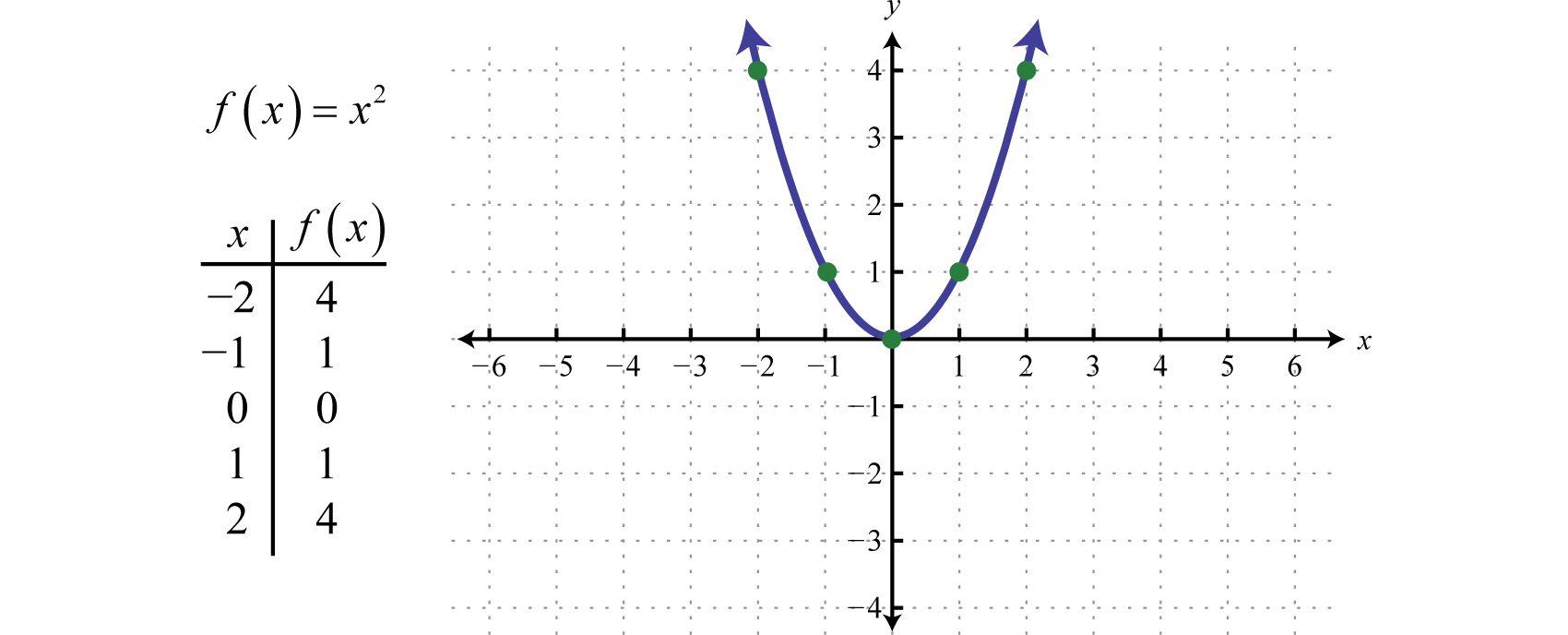This general curved shape is called a parabolaThe U-shaped graph of any quadratic function defined by $f(x)=ax2+bx+c$, where a, b, and c are real numbers and $a≠0.$ and is shared by the graphs of all quadratic functions. Note that the graph is indeed a function as it passes the vertical line test. Furthermore, the domain of this function consists of the set of all real numbers $(−∞,∞)$ and the range consists of the set of nonnegative numbers $[0,∞).$

When graphing parabolas, we want to include certain special points in the graph. The y-intercept is the point where the graph intersects the y-axis. The x-intercepts are the points where the graph intersects the x-axis. The vertexThe point that defines the minimum or maximum of a parabola. is the point that defines the minimum or maximum of the graph. Lastly, the line of symmetryThe vertical line through the vertex, $x=−b2a$, about which the parabola is symmetric. (also called the axis of symmetryA term used when referencing the line of symmetry.) is the vertical line through the vertex, about which the parabola is symmetric.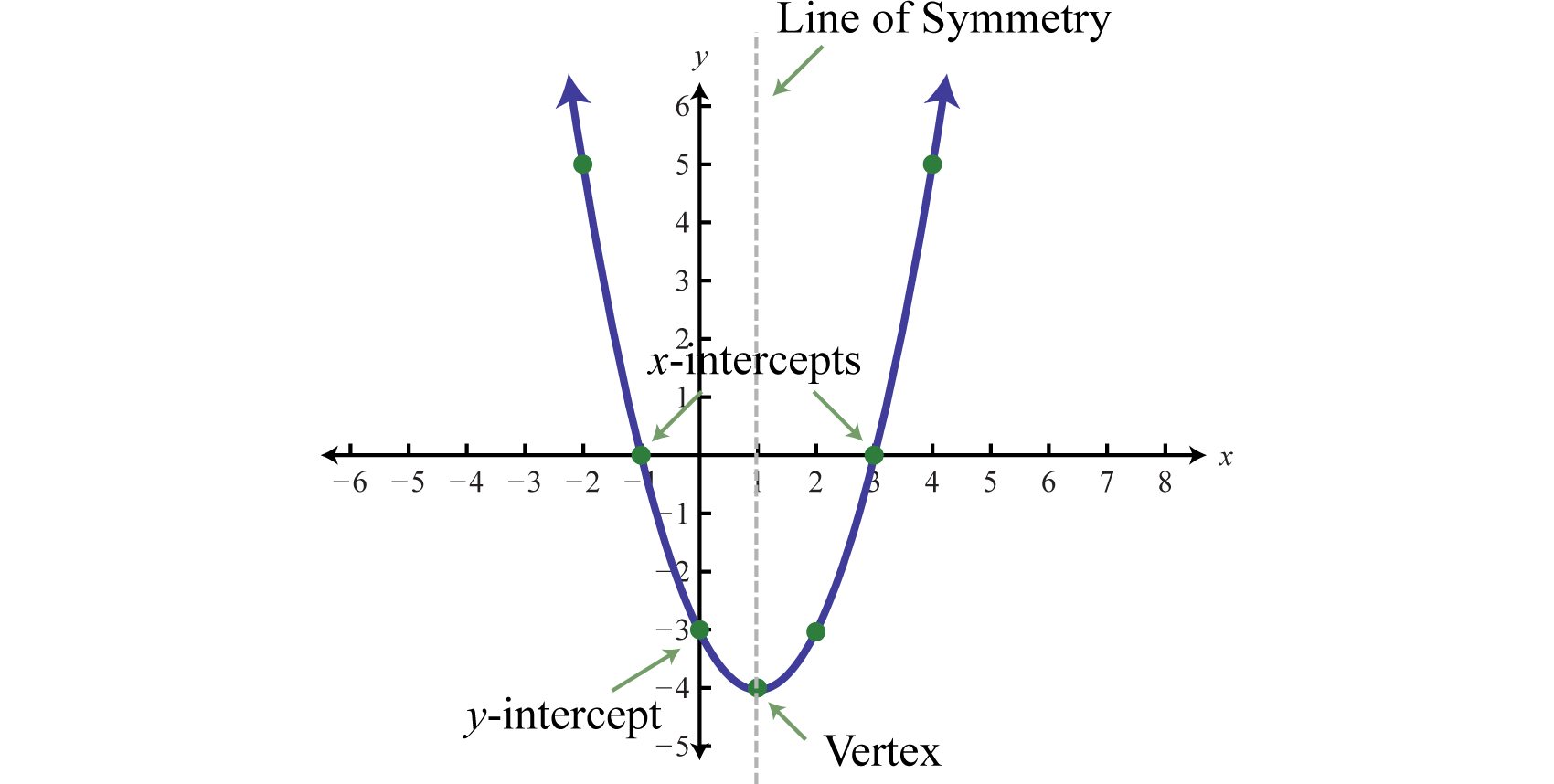For any parabola, we will find the vertex and y-intercept. In addition, if the x-intercepts exist, then we will want to determine those as well. Guessing at the x-values of these special points is not practical; therefore, we will develop techniques that will facilitate finding them. Many of these techniques will be used extensively as we progress in our study of algebra.

Given a quadratic function $f(x)=ax2+bx+c$, find the y-intercept by evaluating the function where $x=0.$ In general, $f(0)=a(0)2+b(0)+c=c$, and we have

$y-intercept (0,c)$

Next, recall that the x-intercepts, if they exist, can be found by setting $f(x)=0.$ Doing this, we have $a2+bx+c=0$, which has general solutions given by the quadratic formula, $x=−b±b2−4ac2a.$ Therefore, the x-intercepts have this general form:

$x-intercepts(−b−b2−4ac2a,0) and (−b+b2−4ac2a,0)$

Using the fact that a parabola is symmetric, we can determine the vertical line of symmetry using the x-intercepts. To do this, we find the x-value midway between the x-intercepts by taking an average as follows:

$x=(−b−b2−4ac2a+−b+b2−4ac2a) ÷2=(−b−b2−4ac−b+b2−4ac2a)÷(21)=−2b2a⋅12=−b2a$

Therefore, the line of symmetry is the vertical line $x=−b2a.$ We can use the line of symmetry to find the the vertex.

$Line of symmetryVertexx=−b2a(−b2a,f(−b2a))$

Generally three points determine a parabola. However, in this section we will find five points so that we can get a better approximation of the general shape. The steps for graphing a parabola are outlined in the following example.

### Example 1

Graph: $f(x)=−x2−2x+3.$

Solution:

Step 1: Determine the y-intercept. To do this, set $x=0$ and find $f(0).$

$f(x)=−x2−2x+3f(0)=−(0)2−2(0)+3=3$

The y-intercept is $(0,3).$

Step 2: Determine the x-intercepts if any. To do this, set $f(x)=0$ and solve for x.

$f(x)=−x2−2x+3Set f(x)=0.0=−x2−2x+3Multiply both sides by −1.0=x2+2x−3Factor.0=(x+3)(x−1)Set each factor equal to zero.x+3=0orx−1=0x=−3x=1$

Here where $f(x)=0$, we obtain two solutions. Hence, there are two x-intercepts, $(−3,0)$ and $(1,0).$

Step 3: Determine the vertex. One way to do this is to first use $x=−b2a$ to find the x-value of the vertex and then substitute this value in the function to find the corresponding y-value. In this example, $a=−1$ and $b=−2.$

$x=−b2a=−(−2)2(−1)=2−2=−1$

Substitute −1 into the original function to find the corresponding y-value.

$f(x)=−x2−2x+3f(−1)=−(−1)2−2(−1)+3=−1+2+3=4$

The vertex is $(−1,4).$

Step 4: Determine extra points so that we have at least five points to plot. Ensure a good sampling on either side of the line of symmetry. In this example, one other point will suffice. Choose $x=−2$ and find the corresponding y-value.

$x yPoint−2 3f(−2)=−(−2)2−2(−2)+3=−4+4+3=3(−2,3)$

Our fifth point is $(−2,3).$

Step 5: Plot the points and sketch the graph. To recap, the points that we have found are

$y-intercept:(0,3)x-intercepts:(−3,0) and (1,0)Vertex:(−1,4)Extra point:(−2,3)$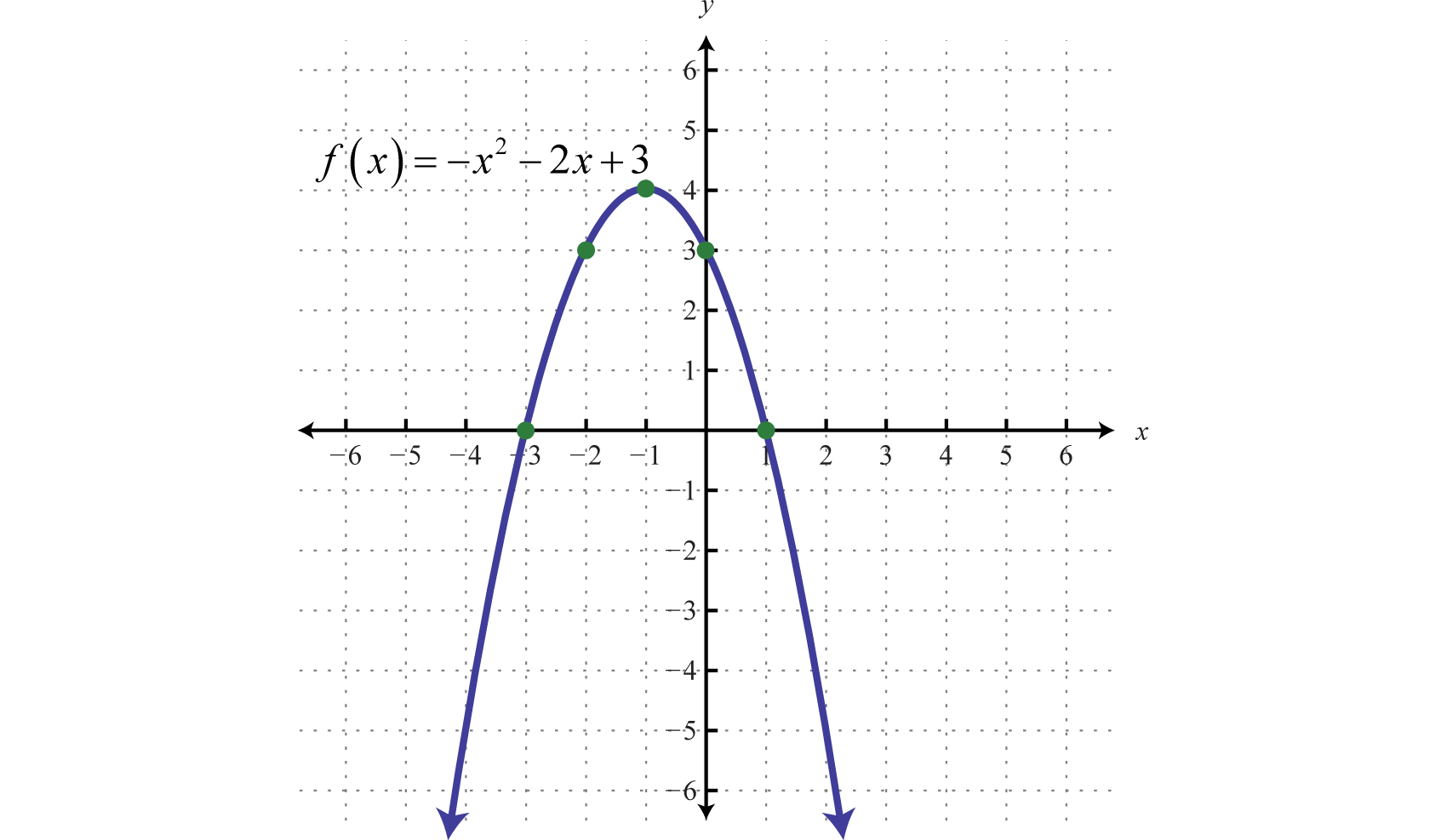The parabola opens downward. In general, use the leading coefficient to determine if the parabola opens upward or downward. If the leading coefficient is negative, as in the previous example, then the parabola opens downward. If the leading coefficient is positive, then the parabola opens upward.All quadratic functions of the form $f(x)=ax2+bx+c$ have parabolic graphs with y-intercept $(0,c).$ However, not all parabolas have x-intercepts.

### Example 2

Graph: $f(x)=2x2+4x+5.$

Solution:

Because the leading coefficient 2 is positive, we note that the parabola opens upward. Here c = 5 and the y-intercept is (0, 5). To find the x-intercepts, set $f(x)=0.$

$f(x)=2x2+4x+50=2x2+4x+5$

In this case, a = 2, b = 4, and c = 5. Use the discriminant to determine the number and type of solutions.

$b2−4ac=(4)2−4(2)(5)=16−40=−24$

Since the discriminant is negative, we conclude that there are no real solutions. Because there are no real solutions, there are no x-intercepts. Next, we determine the x-value of the vertex.

$x=−b2a=−(4)2(2)=−44=−1$

Given that the x-value of the vertex is −1, substitute −1 into the original equation to find the corresponding y-value.

$f(x)=2x2+4x+5f(−1)=2(−1)2+4(−1)+5=2−4+5=3$

The vertex is (−1, 3). So far, we have only two points. To determine three more, choose some x-values on either side of the line of symmetry, x = −1. Here we choose x-values −3, −2, and 1.

$x yPoints−3 11f(−3)=2(−3)2+4(−3)+5=18−12+5=11(−3,11)−2 5f(−2)=2(−2)2+4(−2)+5=8−8+5=5(−2,5)1 11f(1)=2(1)2+4(1)+5=2+4+5=11(1,11)$

To summarize, we have

$y-intercept:(0,5)x-intercepts:NoneVertex:(−1,3)Extra points:(−3,11),(−2,5),(1,11)$

Plot the points and sketch the graph.### Example 3

Graph: $f(x)=x2−2x−1.$

Solution:

Since a = 1, the parabola opens upward. Furthermore, c = −1, so the y-intercept is $(0,−1).$ To find the x-intercepts, set $f(x)=0.$

$f(x)=x2−2x−10=x2−2x−1$

In this case, solve using the quadratic formula with a = 1, b = −2, and c = −1.

$x=−b±b2−4ac2a=−(−2)±(−2)2−4(1)(−1)2(1)=2±82=2±222=2(1±2)2=1±2$

Here we obtain two real solutions for x, and thus there are two x-intercepts:

$(1−2,0)and(1+2,0)Exact values(−0.41,0)(2.41,0)Approximate values$

Approximating the x-intercepts using a calculator will help us plot the points. However, we will present the exact x-intercepts on the graph. Next, find the vertex.

$x=−b2a=−(−2)2(1)=22=1$

Given that the x-value of the vertex is 1, substitute into the original equation to find the corresponding y-value.

$y=x2−2x−1=(1)2−2(1)−1=1−2−1=−2$

The vertex is (1, −2). We need one more point.

$x yPoint2 −1f(2)=(2)2−2(2)−1=4−4−1=−1(2,−1)$

To summarize, we have

$y-intercept:(0,−1)x-intercepts:(1−2,0) and (1+2,0)Vertex:(1,−2)Extra point:(2,−1)$

Plot the points and sketch the graph.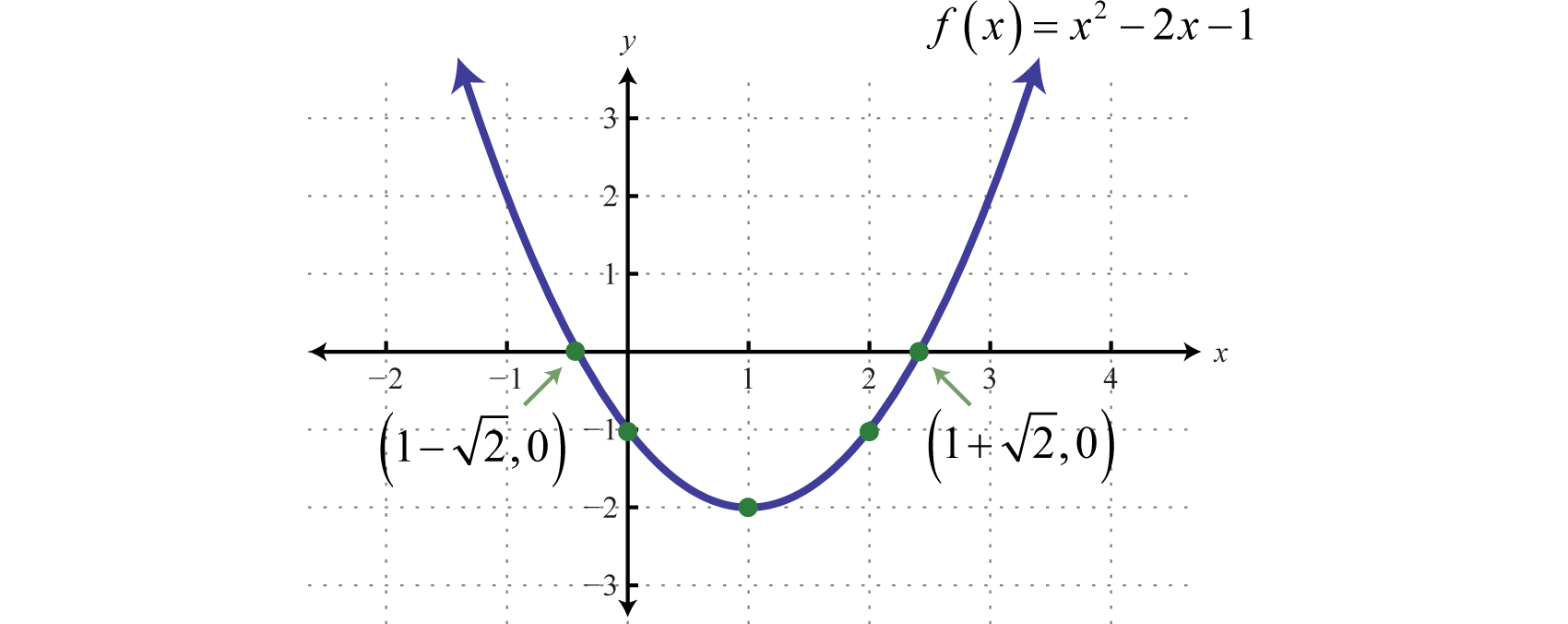Try this! Graph: $g(x)=−4x2+12x−9.$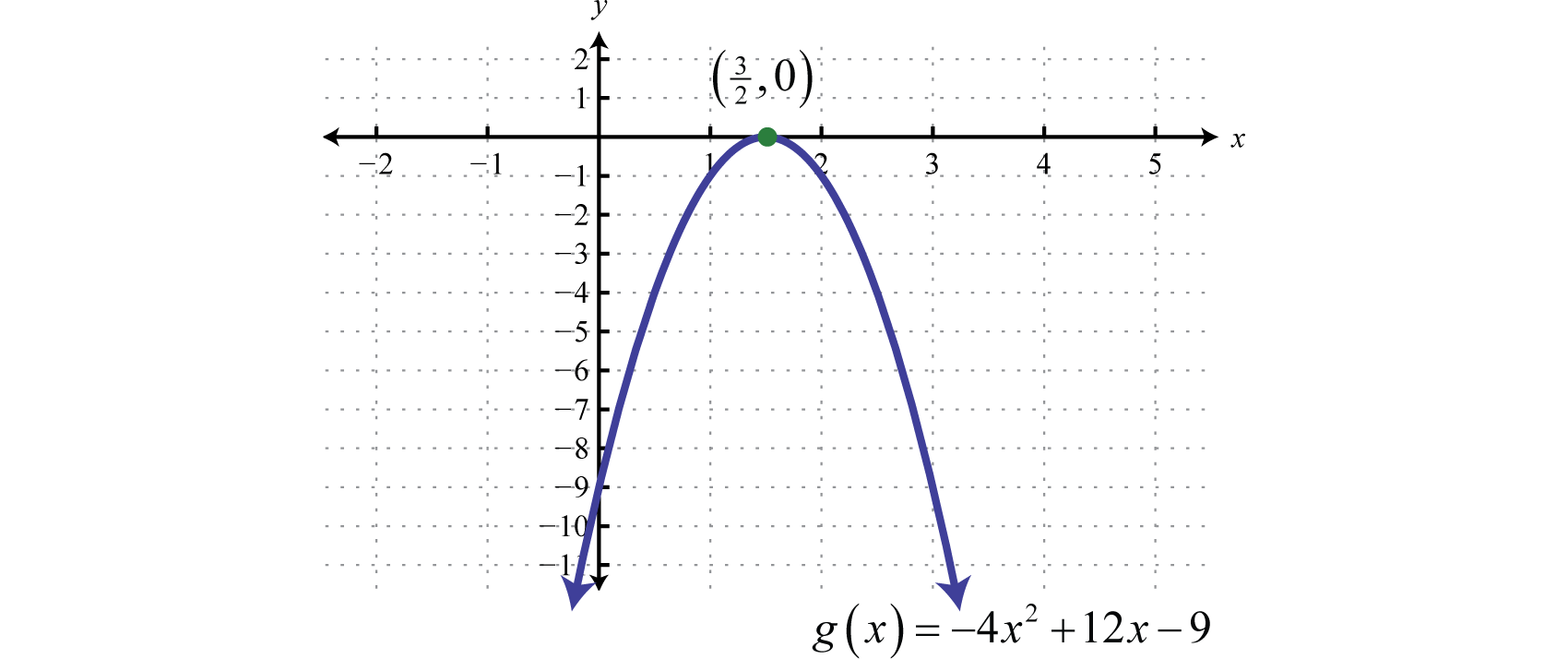## Finding the Maximum or Minimum

It is often useful to find the maximum and/or minimum values of functions that model real-life applications. To find these important values given a quadratic function, we use the vertex. If the leading coefficient a is positive, then the parabola opens upward and there will be a minimum y-value. If the leading coefficient a is negative, then the parabola opens downward and there will be a maximum y-value.

### Example 4

Determine the maximum or minimum: $y=−4x2+24x−35.$

Solution:

Since a = −4, we know that the parabola opens downward and there will be a maximum y-value. To find it, first find the x-value of the vertex.

$x=−b2ax-value of the vertex.=−242(−4)Substitute a = -4 and b = 24.=−24−8Simplify.=3$

The x-value of the vertex is 3. Substitute this value into the original equation to find the corresponding y-value.

$y=−4x2+24x−35Substitute x = 3.=−4(3)2+24(3)−35Simplify.=−36+72−35=1$

The vertex is (3, 1). Therefore, the maximum y-value is 1, which occurs where x = 3, as illustrated below: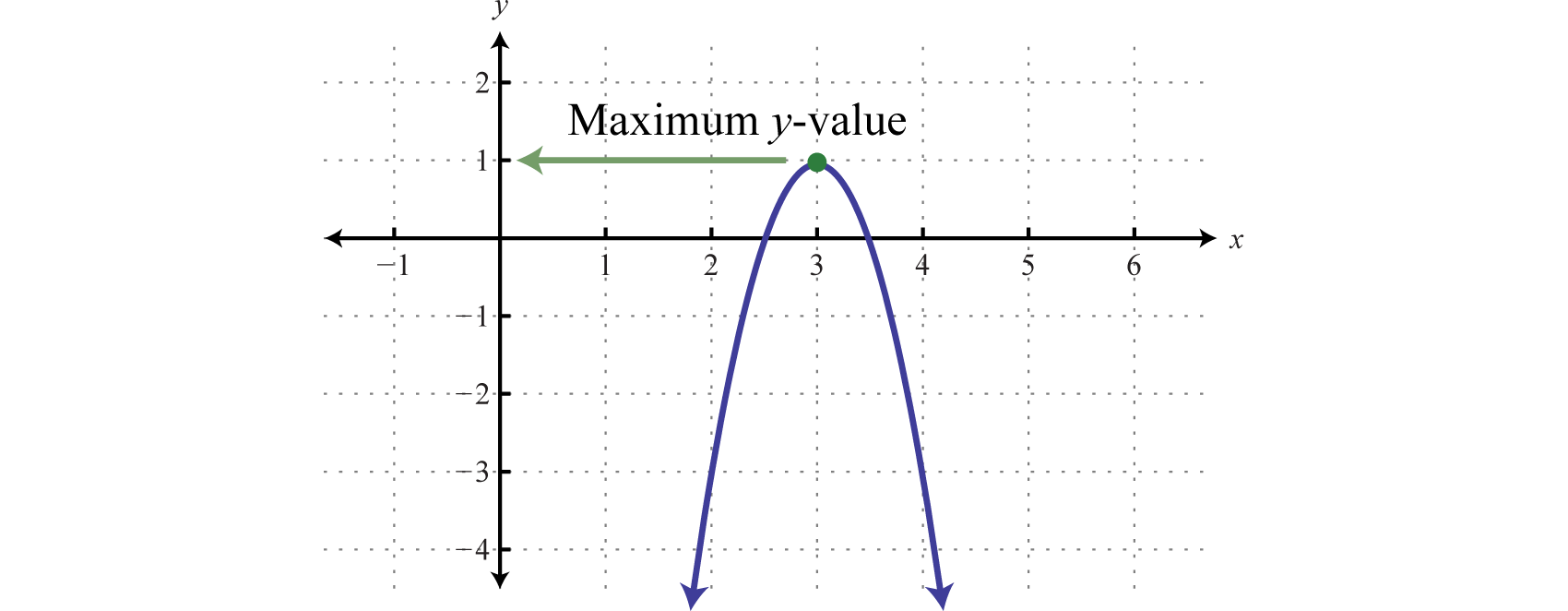Note: The graph is not required to answer this question.

### Example 5

Determine the maximum or minimum: $y=4x2−32x+62.$

Solution:

Since a = 4, the parabola opens upward and there is a minimum y-value. Begin by finding the x-value of the vertex.

$x=−b2a=−−322(4)Substitute a = 4 and b = -32.=−−328Simplify.=4$

Substitute x = 4 into the original equation to find the corresponding y-value.

$y=4x2−32x+62=4(4)2−32(4)+62=64−128+62=−2$

The vertex is (4, −2). Therefore, the minimum y-value of −2 occurs where x = 4, as illustrated below: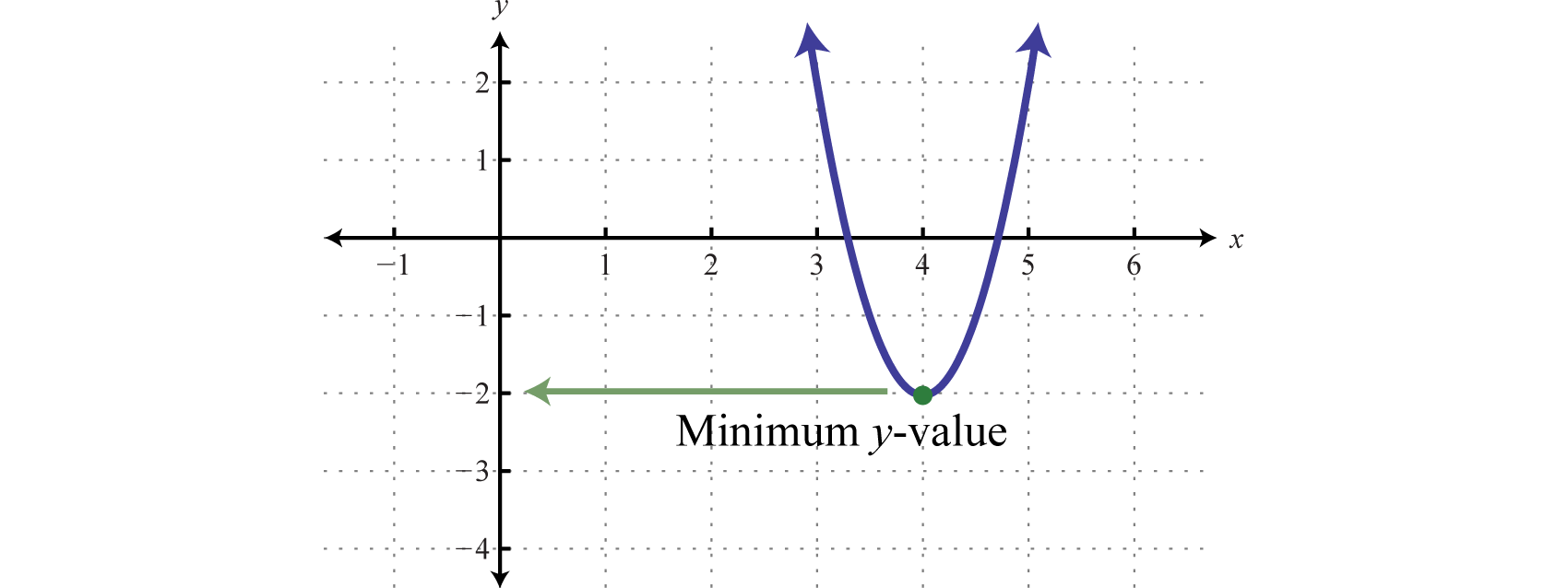### Example 6

The height in feet of a projectile is given by the function $h(t)=−16t2+72t$, where t represents the time in seconds after launch. What is the maximum height reached by the projectile?

Solution:

Here $a=−16$, and the parabola opens downward. Therefore, the y-value of the vertex determines the maximum height. Begin by finding the time at which the vertex occurs.

$t=−b2a=−722(−16)=7232=94$

The maximum height will occur in $94$ seconds (or $214$ seconds). Substitute this time into the function to determine the maximum height attained.

$h(94)=−16(94)2+72(94)=−16(8116)+72(94)=−81+162=81$

Answer: The maximum height of the projectile is 81 feet.

## Finding the Vertex by Completing the Square

In this section, we demonstrate an alternate approach for finding the vertex. Any quadratic function $f(x)=ax2+bx+c$ can be rewritten in vertex formA quadratic function written in the form $f(x)=a(x−h)2+k.$,

$f(x)=a(x−h)2+k$

In this form, the vertex is $(h,k).$ To see that this is the case, consider graphing $f(x)=(x−2)2+3$ using the transformations.

$y=x2Basic squaring functiony=(x−2)2Horizontal shift right 2 unitsy=(x−2)2+3Vertical shift up 3 units$

Use these translations to sketch the graph,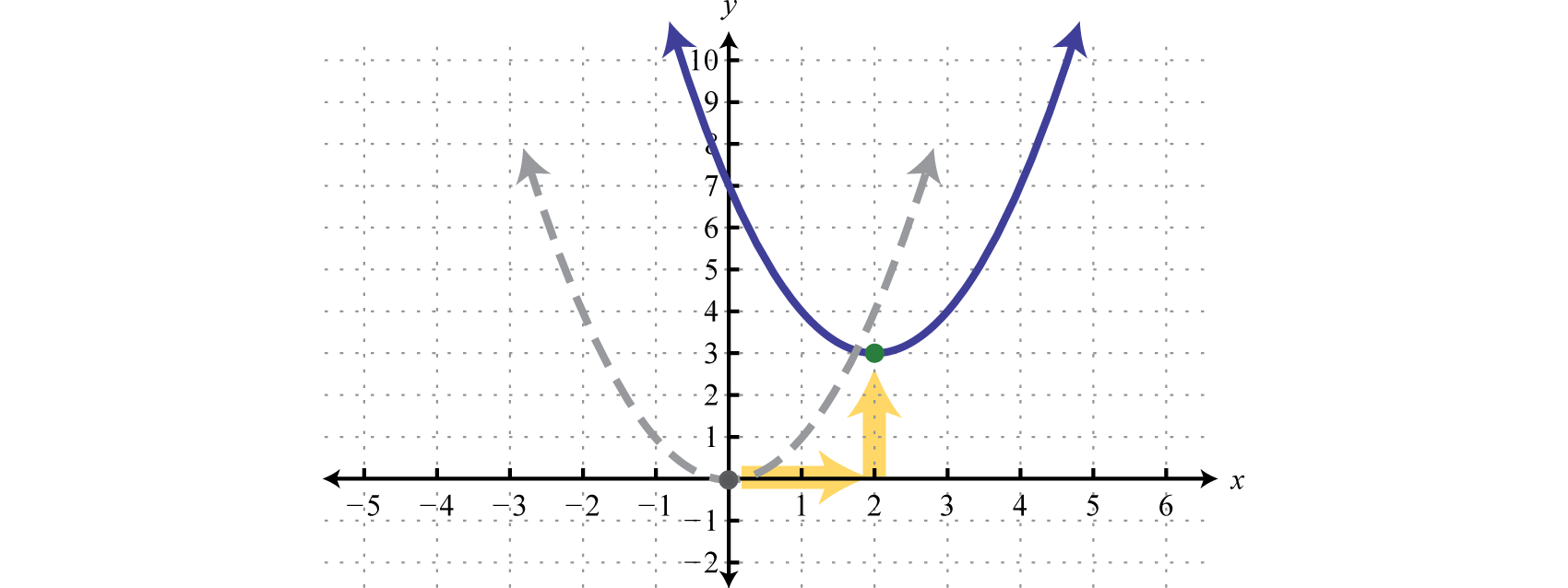Here we can see that the vertex is (2, 3).

$f(x)=a(x−h)2+k↓↓f(x)=(x−2)2+3$

When the equation is in this form, we can read the vertex directly from it.

### Example 7

Determine the vertex: $f(x)=2(x+3)2−2.$

Solution:

Rewrite the equation as follows before determining h and k.

$f(x)=a(x−h)2+k↓↓f(x)=2[x−(−3)]2+(−2)$

Here h = −3 and k = −2.

Answer: The vertex is (−3, −2).

Often the equation is not given in vertex form. To obtain this form, complete the square.

### Example 8

Rewrite in vertex form and determine the vertex: $f(x)=x2+4x+9.$

Solution:

Begin by making room for the constant term that completes the square.

$f(x)=x2+4x+9=x2+4x+___+9−___$

The idea is to add and subtract the value that completes the square, $(b2)2$, and then factor. In this case, add and subtract $(42)2=(2)2=4.$

$f(x)=x2+4x+9Add and subtract 4.=x2+4x +4+9 −4Factor.=(x2+4x+4)+5.=(x+3)(x+2)+5=(x+2)2+5$

Adding and subtracting the same value within an expression does not change it. Doing so is equivalent to adding 0. Once the equation is in this form, we can easily determine the vertex.

$f(x)=a(x−h)2+k↓↓f(x)=(x−(−2))2+5$

Here h = −2 and k = 5.

Answer: The vertex is (−2, 5).

If there is a leading coefficient other than 1, then we must first factor out the leading coefficient from the first two terms of the trinomial.

### Example 9

Rewrite in vertex form and determine the vertex: $f(x)=2x2−4x+8.$

Solution:

Since a = 2, factor this out of the first two terms in order to complete the square. Leave room inside the parentheses to add and subtract the value that completes the square.

$f(x)=2x2−4x+8=2(x2−2x )+8$

Now use −2 to determine the value that completes the square. In this case, $(−22)2=(−1)2=1.$ Add and subtract 1 and factor as follows:

$f(x)=2x2−4x+8=2(x2−2x+__−__)+8Add and subtract 1.=2(x2−2x +1−1)+8 Factor.=2[ (x−1)(x−1)−1 ]+8=2[ (x−1)2−1 ]+8Distribute the 2.=2(x−1)2−2+8=2(x−1)2+6$

In this form, we can easily determine the vertex.

$f(x)=a(x−h)2+k↓↓f(x)=2(x−1)2+6$

Here h = 1 and k = 6.

Answer: The vertex is (1, 6).

Try this! Rewrite in vertex form and determine the vertex: $f(x)=−2x2−12x+3.$

Answer: $f(x)=−2(x+3)2+21$; vertex: $(−3,21)$

### Key Takeaways

• The graph of any quadratic function $f(x)=ax2+bx+c$, where a, b, and c are real numbers and $a≠0$, is called a parabola.
• When graphing a parabola always find the vertex and the y-intercept. If the x-intercepts exist, find those as well. Also, be sure to find ordered pair solutions on either side of the line of symmetry, $x=−b2a.$
• Use the leading coefficient, a, to determine if a parabola opens upward or downward. If a is positive, then it opens upward. If a is negative, then it opens downward.
• The vertex of any parabola has an x-value equal to $−b2a.$ After finding the x-value of the vertex, substitute it into the original equation to find the corresponding y-value. This y-value is a maximum if the parabola opens downward, and it is a minimum if the parabola opens upward.
• The domain of a parabola opening upward or downward consists of all real numbers. The range is bounded by the y-value of the vertex.
• An alternate approach to finding the vertex is to rewrite the quadratic function in the form $f(x)=a(x−h)2+k.$ When in this form, the vertex is $(h,k)$ and can be read directly from the equation. To obtain this form, take $f(x)=ax2+bx+c$ and complete the square.

### Part A: The Graph of Quadratic Functions

Does the parabola open upward or downward? Explain.

1. $y=x2−9x+20$

2. $y=x2−12x+32$

3. $y=−2x2+5x+12$

4. $y=−6x2+13x−6$

5. $y=64−x2$

6. $y=−3x+9x2$

Determine the x- and y-intercepts.

1. $y=x2+4x−12$

2. $y=x2−13x+12$

3. $y=2x2+5x−3$

4. $y=3x2−4x−4$

5. $y=−5x2−3x+2$

6. $y=−6x2+11x−4$

7. $y=4x2−27$

8. $y=9x2−50$

9. $y=x2−x+1$

10. $y=x2−6x+4$

Find the vertex and the line of symmetry.

1. $y=−x2+10x−34$

2. $y=−x2−6x+1$

3. $y=−4x2+12x−7$

4. $y=−9x2+6x+2$

5. $y=4x2−1$

6. $y=x2−16$

Graph. Find the vertex and the y-intercept. In addition, find the x-intercepts if they exist.

1. $f(x)=x2−2x−8$

2. $f(x)=x2−4x−5$

3. $f(x)=−x2+4x+12$

4. $f(x)=−x2−2x+15$

5. $f(x)=x2−10x$

6. $f(x)=x2+8x$

7. $f(x)=x2−9$

8. $f(x)=x2−25$

9. $f(x)=1−x2$

10. $f(x)=4−x2$

11. $f(x)=x2−2x+1$

12. $f(x)=x2+4x+4$

13. $f(x)=−4x2+12x−9$

14. $f(x)=−4x2−4x+3$

15. $f(x)=x2−2$

16. $f(x)=x2−3$

17. $f(x)=−4x2+4x−3$

18. $f(x)=4x2+4x+3$

19. $f(x)=x2−2x−2$

20. $f(x)=x2−6x+6$

21. $f(x)=−2x2+6x−3$

22. $f(x)=−4x2+4x+1$

23. $f(x)=x2+3x+4$

24. $f(x)=−x2+3x−4$

25. $f(x)=−2x2+3$

26. $f(x)=−2x2−1$

27. $f(x)=2x2+4x−3$

28. $f(x)=3x2+2x−2$

### Part B: Finding the Maximum or Minimum

Determine the maximum or minimum y-value.

1. $y=−x2−6x+1$

2. $y=−x2−4x+8$

3. $y=25x2−10x+5$

4. $y=16x2−24x+7$

5. $y=−x2$

6. $y=1−9x2$

7. $y=20x−10x2$

8. $y=12x+4x2$

9. $y=3x2−4x−2$

10. $y=6x2−8x+5$

11. $y=x2−5x+1$

12. $y=1−x−x2$

Given the following quadratic functions, determine the domain and range.

1. $f(x)=3x2+30x+50$

2. $f(x)=5x2−10x+1$

3. $g(x)=−2x2+4x+1$

4. $g(x)=−7x2−14x−9$

5. $f(x)=x2+x−1$

6. $f(x)=−x2+3x−2$

7. The height in feet reached by a baseball tossed upward at a speed of 48 feet per second from the ground is given by the function $h(t)=−16t2+48t$, where t represents the time in seconds after the ball is thrown. What is the baseball’s maximum height and how long does it take to attain that height?

8. The height in feet of a projectile launched straight up from a mound is given by the function $h(t)=−16t2+96t+4$, where t represents seconds after launch. What is the maximum height?

9. The profit in dollars generated by producing and selling x custom lamps is given by the function $P(x)=−10x2+800x−12,000.$ What is the maximum profit?

10. The profit in dollars generated from producing and selling a particular item is modeled by the formula $P(x)=100x−0.0025x2$, where x represents the number of units produced and sold. What number of units must be produced and sold to maximize revenue?

11. The average number of hits to a radio station Web site is modeled by the formula $f(x)=450t2−3,600t+8,000$, where t represents the number of hours since 8:00 a.m. At what hour of the day is the number of hits to the Web site at a minimum?

12. The value in dollars of a new car is modeled by the formula $V(t)=125t2−3,000t+22,000$, where t represents the number of years since it was purchased. Determine the minimum value of the car.

13. The daily production cost in dollars of a textile manufacturing company producing custom uniforms is modeled by the formula $C(x)=0.02x2−20x+10,000$, where x represents the number of uniforms produced.

1. How many uniforms should be produced to minimize the daily production costs?
2. What is the minimum daily production cost?
14. The area in square feet of a certain rectangular pen is given by the formula $A=14w−w2$, where w represents the width in feet. Determine the width that produces the maximum area.

### Part C: Finding the Vertex by Completing the Square

Determine the vertex.

1. $y=−(x−5)2+3$

2. $y=−2(x−1)2+7$

3. $y=5(x+1)2+6$

4. $y=3(x+4)2+10$

5. $y=−5(x+8)2−1$

6. $y=(x+2)2−5$

Rewrite in vertex form $y=a(x−h)2+k$ and determine the vertex.

1. $y=x2−14x+24$

2. $y=x2−12x+40$

3. $y=x2+4x−12$

4. $y=x2+6x−1$

5. $y=2x2−12x−3$

6. $y=3x2−6x+5$

7. $y=−x2+16x+17$

8. $y=−x2+10x$

Graph. Find the vertex and the y-intercept. In addition, find the x-intercepts if they exist.

1. $f(x)=x2−1$

2. $f(x)=x2+1$

3. $f(x)=(x−1)2$

4. $f(x)=(x+1)2$

5. $f(x)=(x−4)2−9$

6. $f(x)=(x−1)2−4$

7. $f(x)=−2(x+1)2+8$

8. $f(x)=−3(x+2)2+12$

9. $f(x)=−5(x−1)2$

10. $f(x)=−(x+2)2$

11. $f(x)=−4(x−1)2−2$

12. $f(x)=9(x+1)2+2$

13. $f(x)=(x+5)2−15$

14. $f(x)=2(x−5)2−3$

15. $f(x)=−2(x−4)2+22$

16. $f(x)=2(x+3)2−13$

### Part D: Discussion Board

1. Write down your plan for graphing a parabola on an exam. What will you be looking for and how will you present your answer? Share your plan on the discussion board.

2. Why is any parabola that opens upward or downward a function? Explain to a classmate how to determine the domain and range.

3. Research and discuss ways of finding a quadratic function that has a graph passing through any three given points. Share a list of steps as well as an example of how to do this.

1. Upward

2. Downward

3. Downward

4. x-intercepts: (−6, 0), (2, 0);y-intercept: (0, −12)

5. x-intercepts: (−3, 0), $(12, 0)$; y-intercept: (0, −3)

6. x-intercepts: (−1, 0), $(25, 0)$; y-intercept: (0, 2)

7. x-intercepts: $(−332,0)$, $(332,0)$; y-intercept: (0, −27)

8. x-intercepts: none; y-intercept: (0, 1)

9. Vertex: (5, −9); line of symmetry: $x=5$

10. Vertex: $(32, 2)$; line of symmetry: $x=32$

11. Vertex: (0, −1); line of symmetry: $x=0$

12.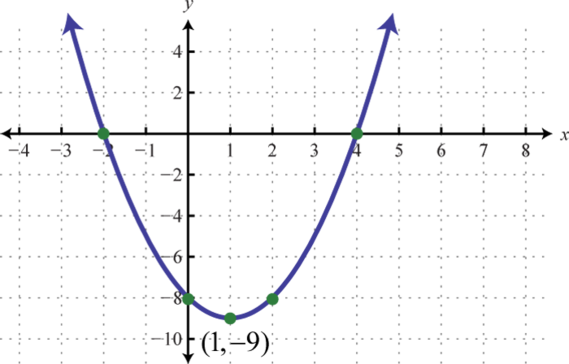13.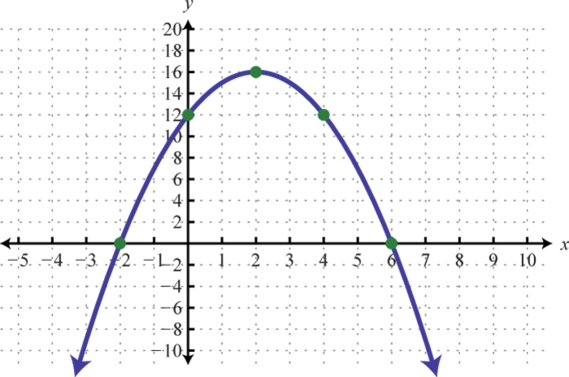14.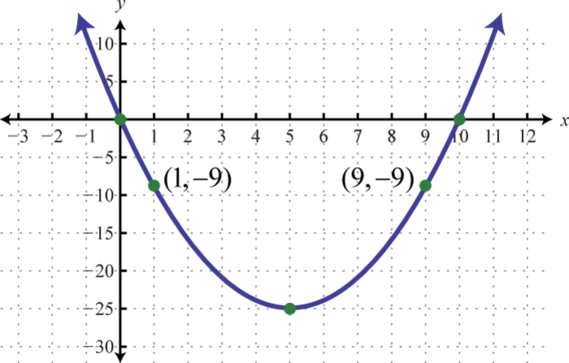15.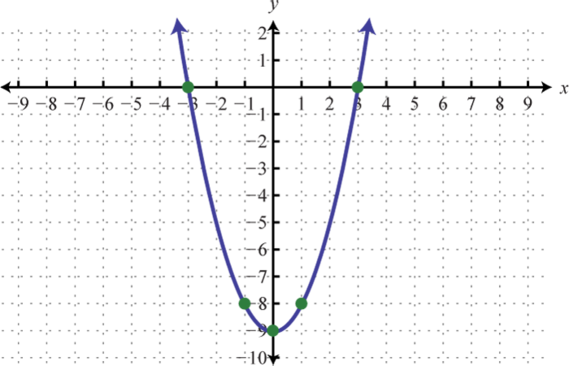16.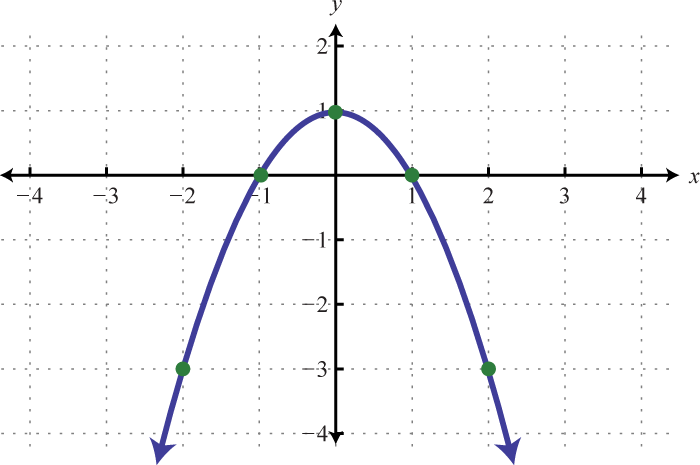17.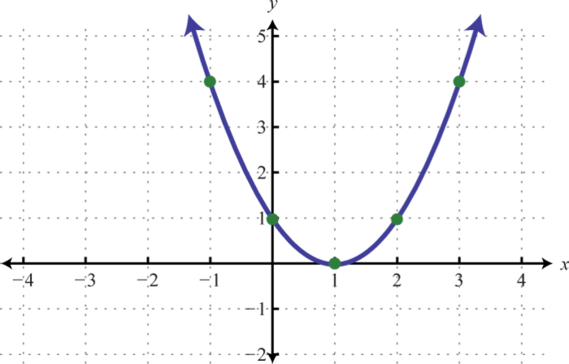18.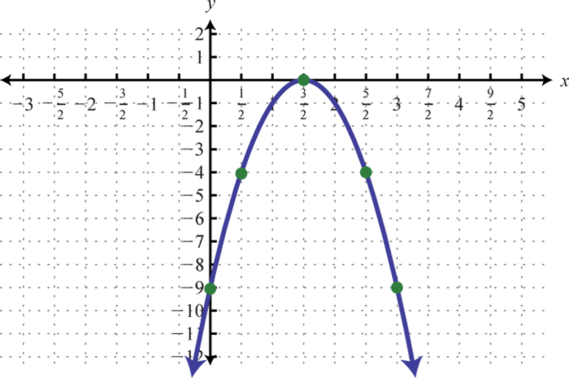19.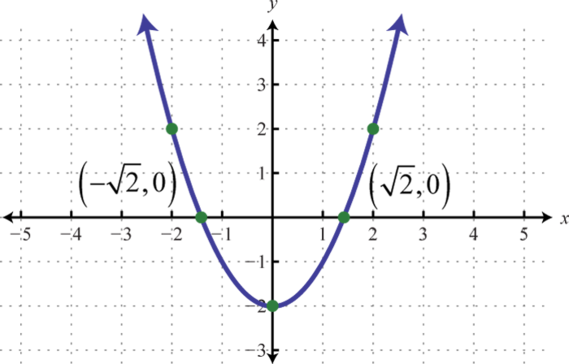20.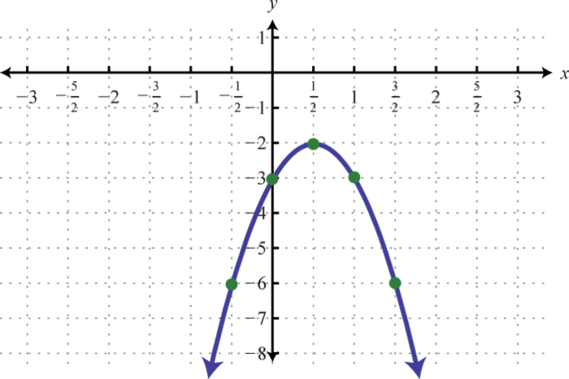21.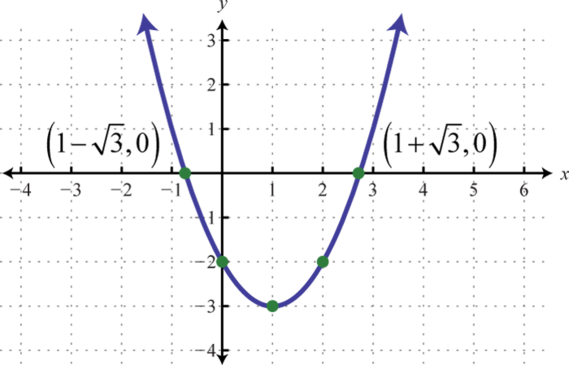22.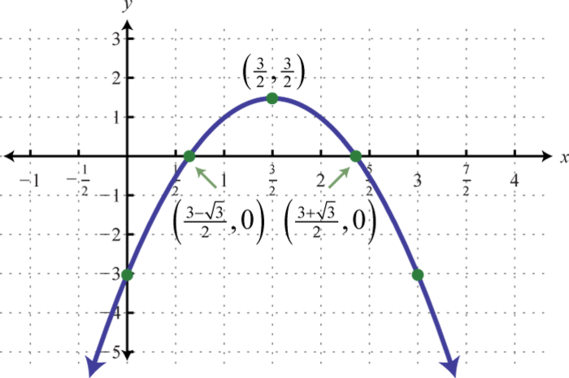23.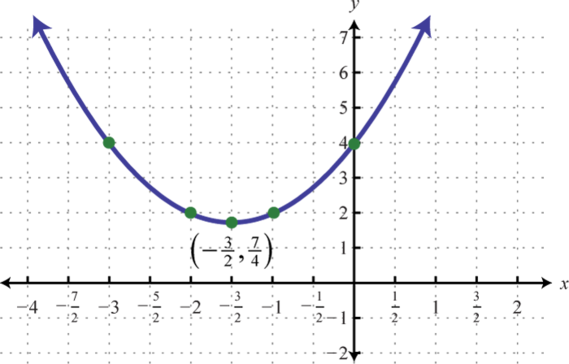24.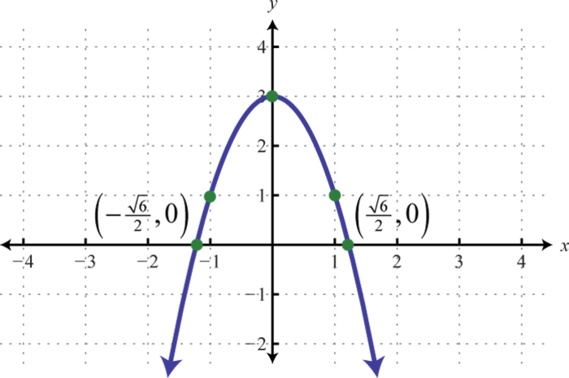25.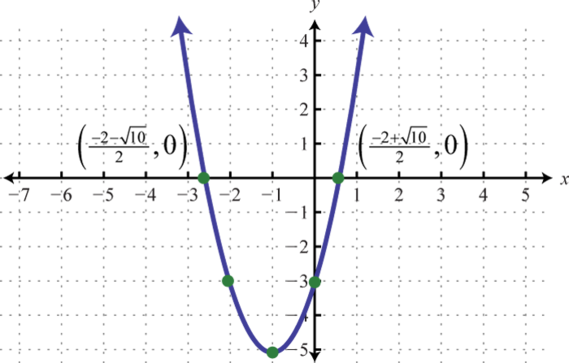1. Maximum: y = 10

2. Minimum: y = 4

3. Maximum: y = 0

4. Maximum: y = 10

5. Minimum: $y=−103$

6. Minimum: $y=−214$

7. Domain: $(−∞,∞)$; range: $[−25,∞)$

8. Domain: $(−∞,∞)$; range: $(−∞,3]$

9. Domain: $(−∞,∞)$; range: $[−54,∞)$

10. The maximum height of 36 feet occurs after 1.5 seconds.

11. \$4,000

12. 12:00 p.m.

1. 500 uniforms
2. \$5,000
1. (5, 3)

2. (−1, 6)

3. (−8, −1)

4. $y=(x−7)2−25$; vertex: (7, −25)

5. $y=(x+2)2−16$; vertex: (−2, −16)

6. $y=2(x−3)2−21$; vertex: (3, −21)

7. $y=−(x−8)2+81$; vertex: (8, 81)

8.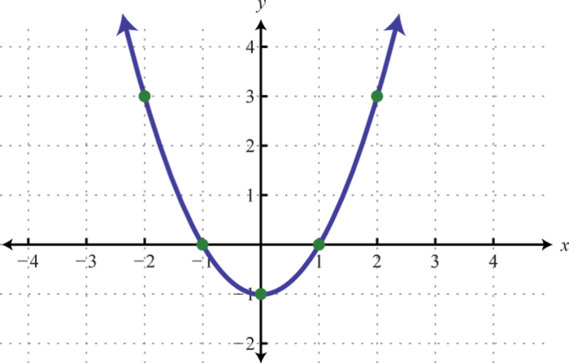9.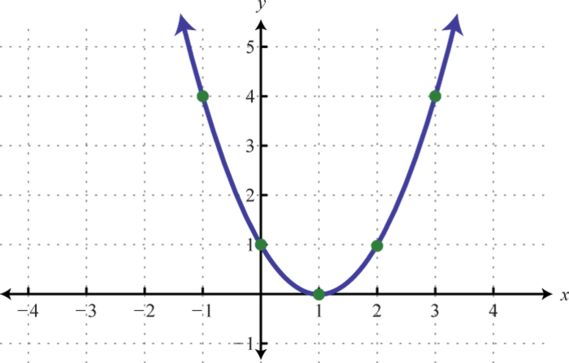10.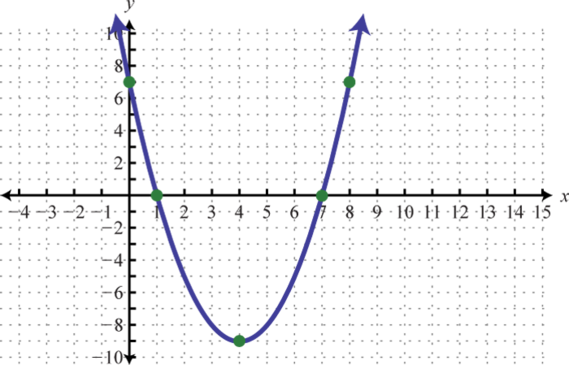11.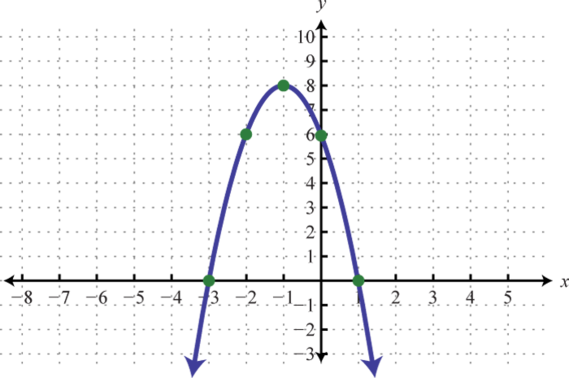12.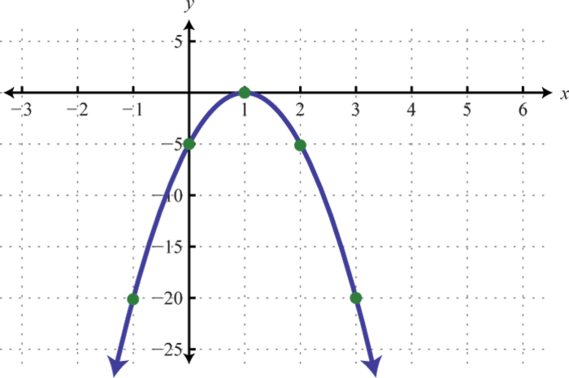13.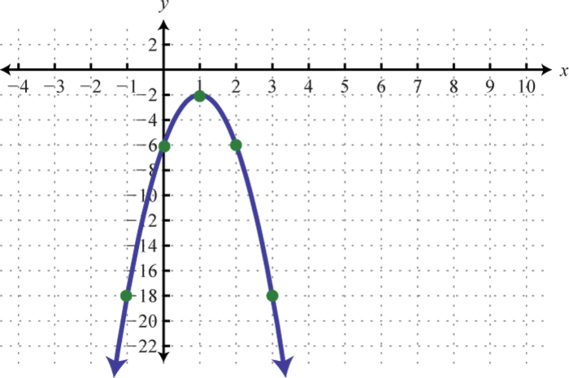14.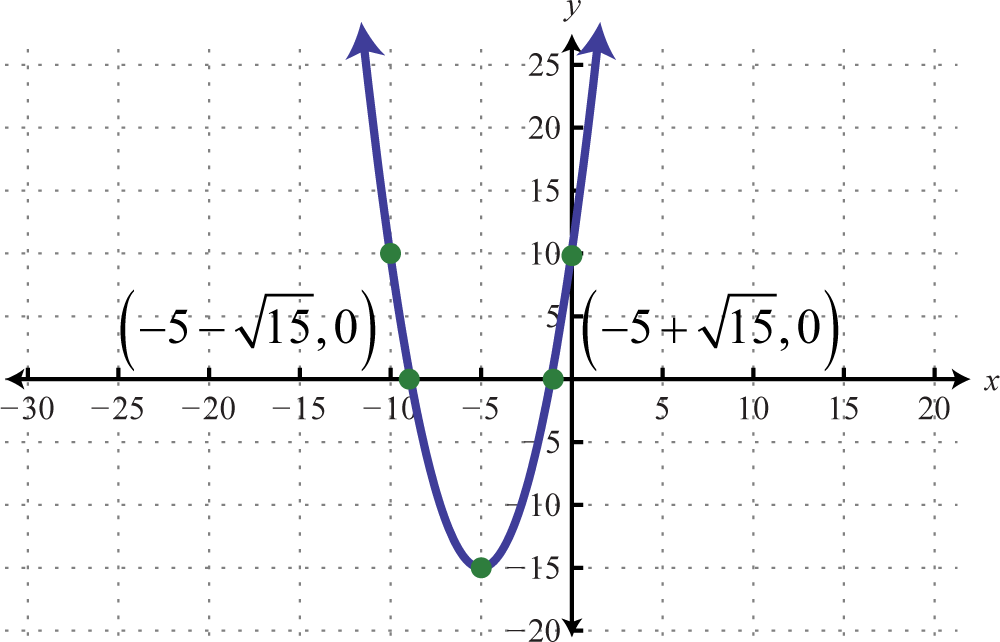15.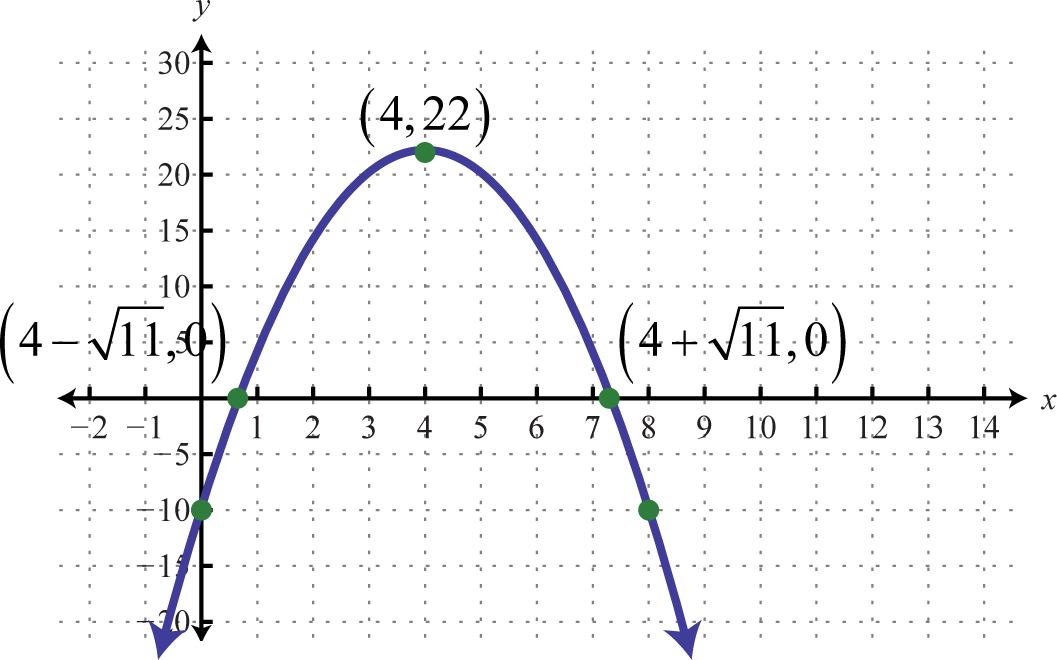### Learning Objectives

1. Check solutions to quadratic inequalities with one variable.
2. Understand the geometric relationship between solutions to quadratic inequalities and their graphs.

A quadratic inequalityA mathematical statement that relates a quadratic expression as either less than or greater than another. is a mathematical statement that relates a quadratic expression as either less than or greater than another. Some examples of quadratic inequalities solved in this section follow.

 $x2−2x−11≤0$ $2x2−7x+3>0$ $9−x2>0$

A solution to a quadratic inequality is a real number that will produce a true statement when substituted for the variable.

### Example 1

Are −3, −2, and −1 solutions to $x2−x−6≤0$?

Solution:

Substitute the given value in for x and simplify.

$x2−x−6≤0x2−x−6≤0x2−x−6≤0(−3)2−(−3)−6≤0(−2)2−(−2)−6≤0(−1)2−(−1)−6≤09+3−6≤04+2−6≤01+1−6≤06≤0✗0≤0✓−4≤0✓$

Answer: −2 and −1 are solutions and −3 is not.

Quadratic inequalities can have infinitely many solutions, one solution, or no solution. If there are infinitely many solutions, graph the solution set on a number line and/or express the solution using interval notation. Graphing the function defined by $f(x)=x2−x−6$ found in the previous example we haveThe result of evaluating for any x-value will be negative, zero, or positive.

$f(−3)=6Positivef(x)>0f(−2)=0Zerof(x)=0f(−1)=−4Negativef(x)<0$

The values in the domain of a function that separate regions that produce positive or negative results are called critical numbersThe values in the domain of a function that separate regions that produce positive or negative results.. In the case of a quadratic function, the critical numbers are the roots, sometimes called the zeros. For example, $f(x)=x2−x−6=(x+2)(x−3)$ has roots −2 and 3. These values bound the regions where the function is positive (above the x-axis) or negative (below the x-axis).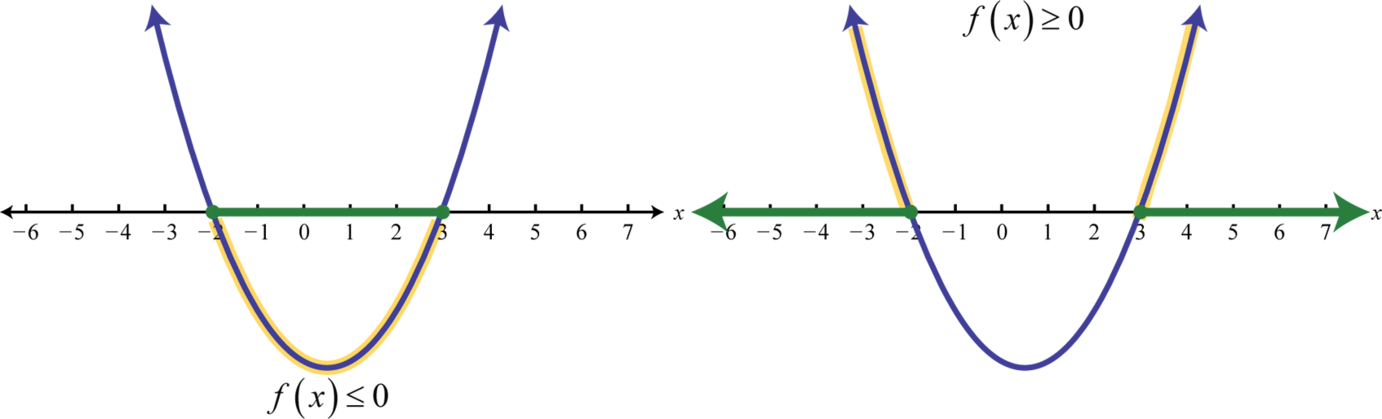Therefore $x2−x−6≤0$ has solutions where $−2≤x≤3$, using interval notation $[−2,3].$ Furthermore, $x2−x−6≥0$ has solutions where $x≤−2$ or $x≥3$, using interval notation $(−∞,−2]∪[−3,∞).$

### Example 2

Given the graph of $f$ determine the solutions to $f(x)>0$: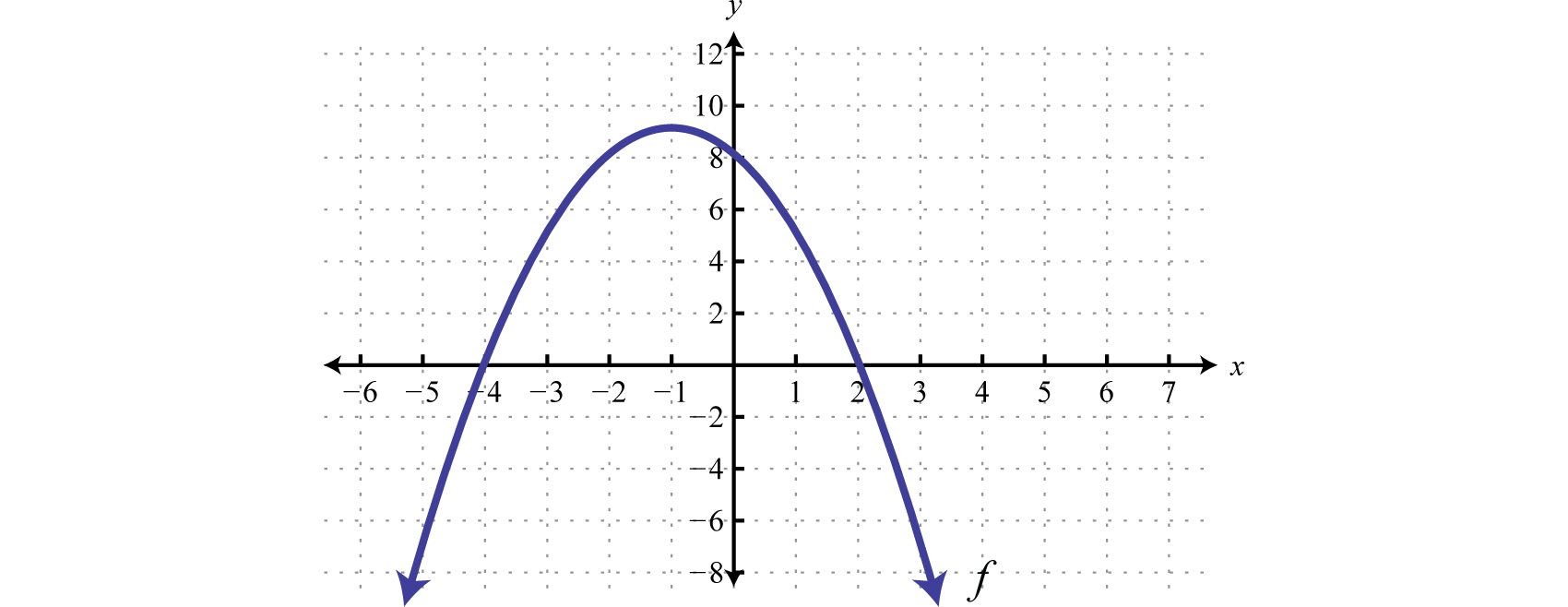Solution:

From the graph we can see that the roots are −4 and 2. The graph of the function lies above the x-axis ($f(x)>0$) in between these roots.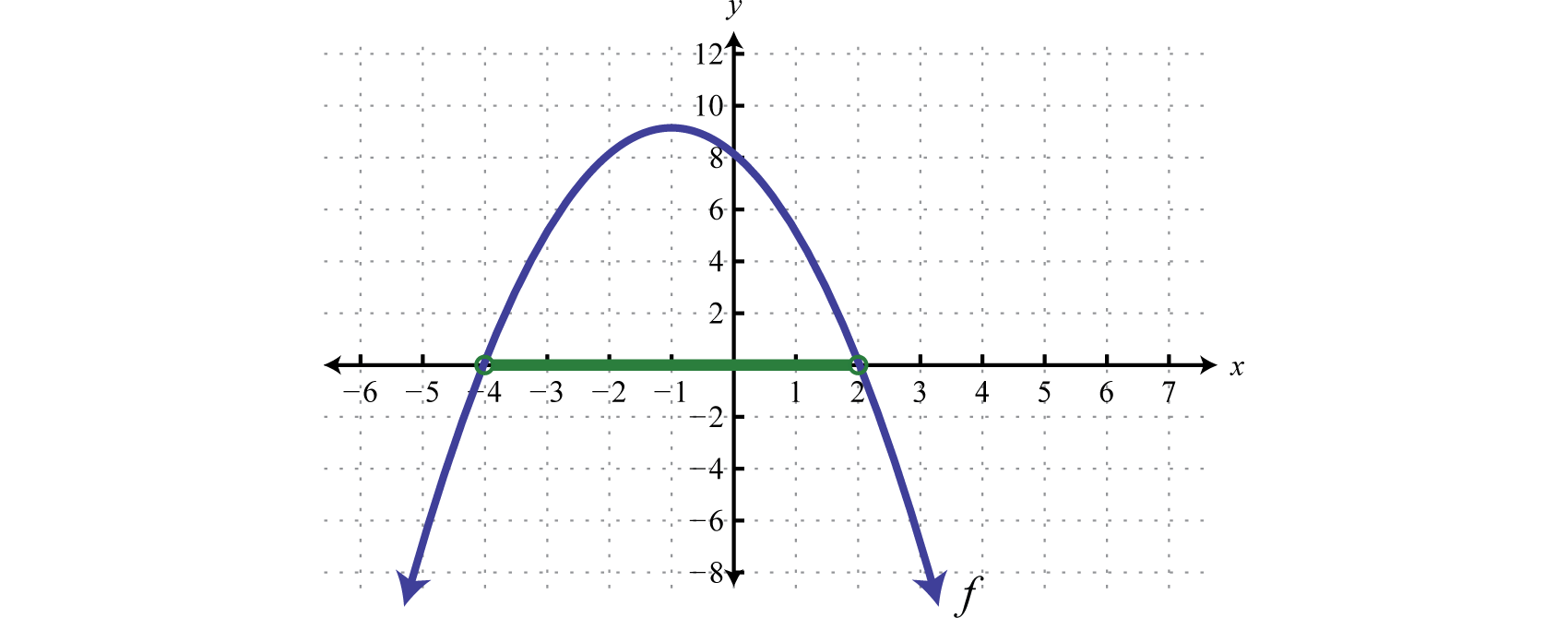Because of the strict inequality, the solution set is shaded with an open dot on each of the boundaries. This indicates that these critical numbers are not actually included in the solution set. This solution set can be expressed two ways,

${x|−4

In this textbook, we will continue to present answers in interval notation.

Answer: $(−4,2)$

Try this! Given the graph of $f$ determine the solutions to $f(x)<0$: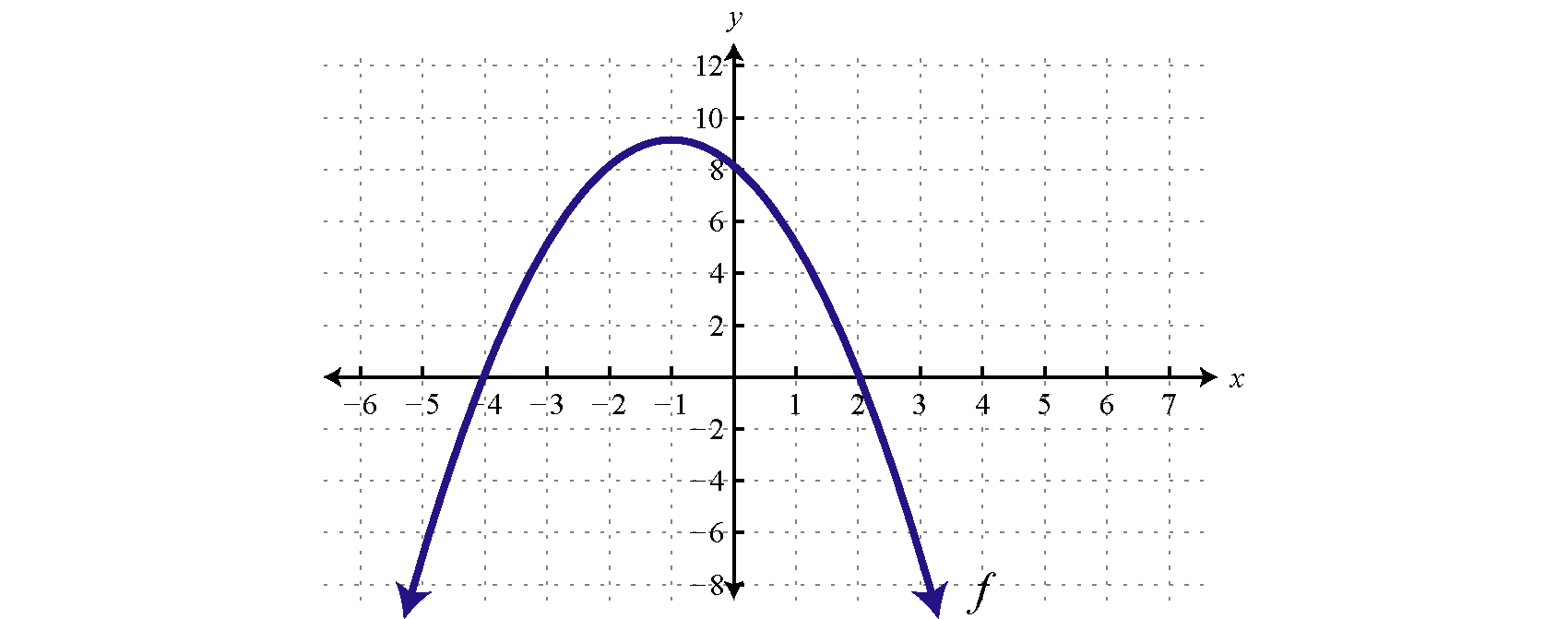Answer: $(−∞,−4)∪(2,∞)$

Next we outline a technique used to solve quadratic inequalities without graphing the parabola. To do this we make use of a sign chartA model of a function using a number line and signs (+ or −) to indicate regions in the domain where the function is positive or negative. which models a function using a number line that represents the x-axis and signs (+ or −) to indicate where the function is positive or negative. For example,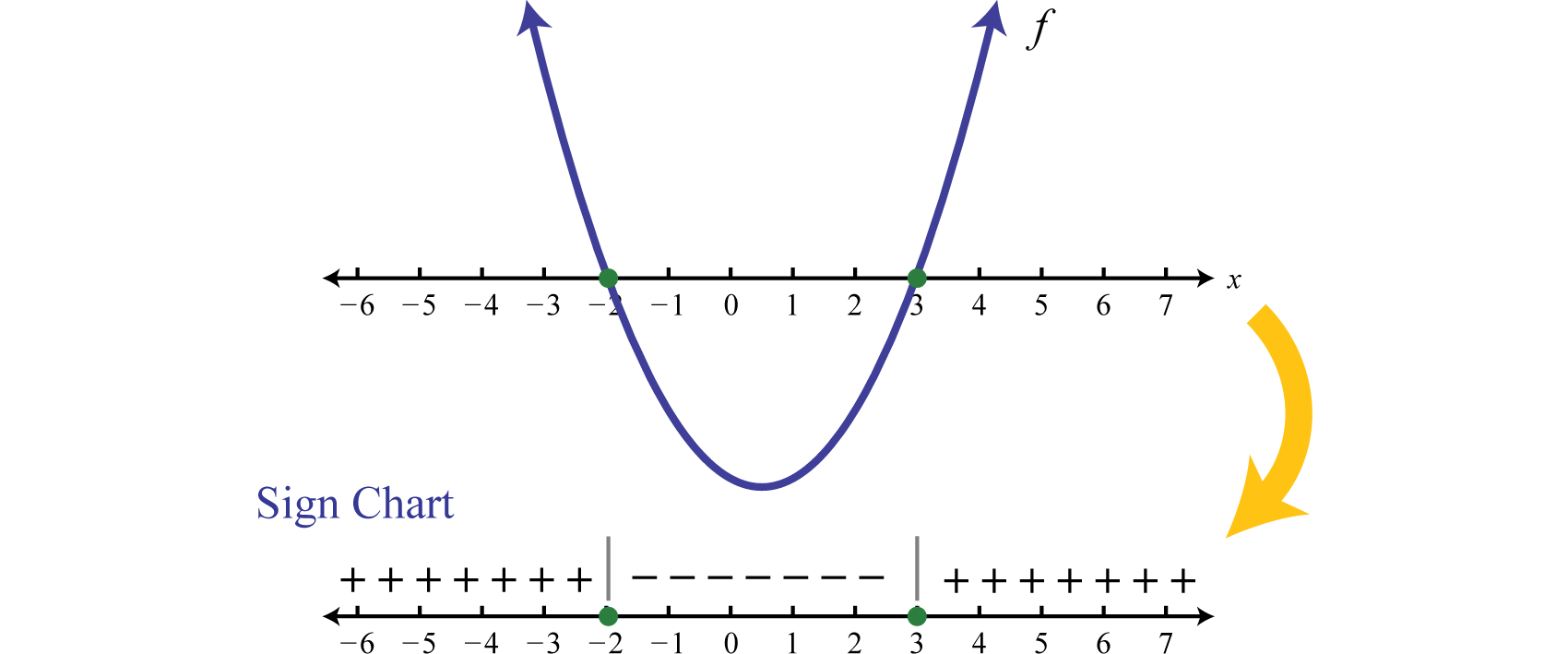The plus signs indicate that the function is positive on the region. The negative signs indicate that the function is negative on the region. The boundaries are the critical numbers, −2 and 3 in this case. Sign charts are useful when a detailed picture of the graph is not needed and are used extensively in higher level mathematics. The steps for solving a quadratic inequality with one variable are outlined in the following example.

### Example 3

Solve: $−x2+6x+7≥0.$

Solution:

It is important to note that this quadratic inequality is in standard form, with zero on one side of the inequality.

Step 1: Determine the critical numbers. For a quadratic inequality in standard form, the critical numbers are the roots. Therefore, set the function equal to zero and solve.

$−x2+6x+7=0−(x2−6x−7)=0−(x+1)(x−7)=0x+1=0orx−7=0x=−1x=7$

The critical numbers are −1 and 7.

Step 2: Create a sign chart. Since the critical numbers bound the regions where the function is positive or negative, we need only test a single value in each region. In this case the critical numbers partition the number line into three regions and we choose test values $x=−3$, $x=0$, and $x=10.$Test values may vary. In fact, we need only determine the sign (+ or −) of the result when evaluating $f(x)=−x2+6x+7=−(x+1)(x−7).$ Here we evaluate using the factored form.

$f(−3)=−(−3+1)(−3−7)=−(−2)(−10)=−Negativef(0)=−(0+1)(0−7)=−(1)(−7)=+Positivef(10)=−(10+1)(10−7)=−(11)(3)=−Negative$

Since the result of evaluating for −3 was negative, we place negative signs above the first region. The result of evaluating for 0 was positive, so we place positive signs above the middle region. Finally, the result of evaluating for 10 was negative, so we place negative signs above the last region, and the sign chart is complete.Step 3: Use the sign chart to answer the question. In this case, we are asked to determine where $f(x)≥0$, or where the function is positive or zero. From the sign chart we see this occurs when x-values are inclusively between −1 and 7.Using interval notation, the shaded region is expressed as $[−1,7].$ The graph is not required; however, for the sake of completeness it is provided below.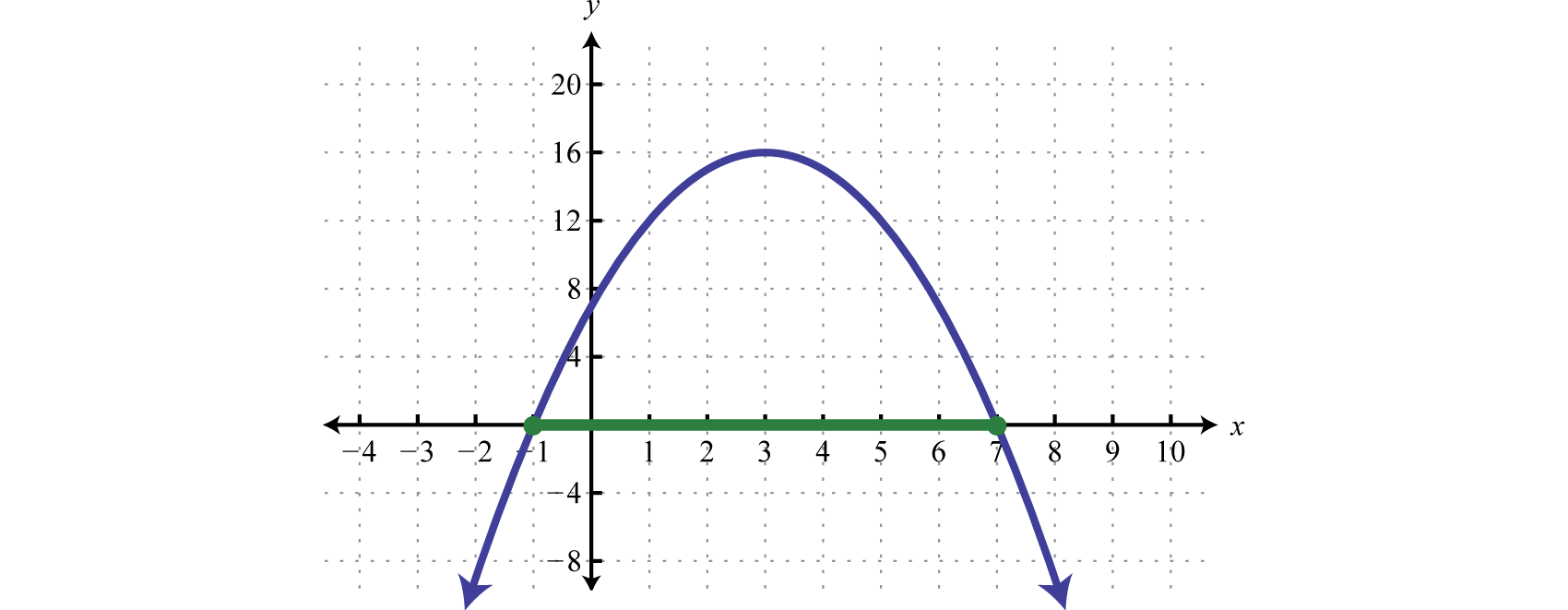Indeed the function is greater than or equal to zero, above or on the x-axis, for x-values in the specified interval.

Answer: $[−1,7]$

### Example 4

Solve: $2x2−7x+3>0.$

Solution:

Begin by finding the critical numbers, in this case, the roots of $f(x)=2x2−7x+3.$

$2x2−7x+3=0(2x−1)(x−3)=02x−1=0orx−3=02x=1x=3x=12$

The critical numbers are $12$ and 3. Because of the strict inequality > we will use open dots.Next choose a test value in each region and determine the sign after evaluating $f(x)=2x2−7x+3=(2x−1)(x−3).$ Here we choose test values −1, 2, and 5.

$f(−1)=[2(−1)−1](−1−3)=(−)(−)=+f(2)=[2(2)−1](2−3)=(+)(−)=−f(5)=[2(5)−1](5−3)=(+)(+)=+$

And we can complete the sign chart.The question asks us to find the x-values that produce positive results (greater than zero). Therefore, shade in the regions with a + over them. This is the solution set.Answer: $(−∞,12)∪(3,∞)$

Sometimes the quadratic function does not factor. In this case we can make use of the quadratic formula.

### Example 5

Solve: $x2−2x−11≤0.$

Solution:

Find the critical numbers.

$x2−2x−11=0$

Identify a, b, and c for use in the quadratic formula. Here $a=1$, $b=−2$, and $c=−11.$ Substitute the appropriate values into the quadratic formula and then simplify.

$x=−b±b2−4ac2a=−(−2)±(−2)2−4(1)(−11)2(1)=2±482=2±432=1±23$

Therefore the critical numbers are $1−23≈−2.5$ and $1+23≈4.5.$ Use a closed dot on the number to indicate that these values will be included in the solution set.Here we will use test values −5, 0, and 7.

$f(−5)=(−5)2−2(−5)−11=25+10−11=+f(0)=(0)2−2(0)−11=0+0−11=−f(7)=(7)2−2(7)−11=49−14−11=+$

After completing the sign chart shade in the values where the function is negative as indicated by the question ($f(x)≤0$).Answer: $[1−23,1+23]$

Try this! Solve: $9−x2>0.$

Answer: $(−3,3)$

It may be the case that there are no critical numbers.

### Example 6

Solve: $x2−2x+3>0.$

Solution:

To find the critical numbers solve,

$x2−2x+3=0$

Substitute $a=1$, $b=−2$, and $c=3$ into the quadratic formula and then simplify.

$x=−b±b2−4ac2a=−(−2)±(−2)2−4(1)(3)2(1)=2±−82=2±2i22=1+i2$

Because the solutions are not real, we conclude there are no real roots; hence there are no critical numbers. When this is the case, the graph has no x-intercepts and is completely above or below the x-axis. We can test any value to create a sign chart. Here we choose $x=0.$

$f(0)=(0)2−2(0)+3=+$

Because the test value produced a positive result the sign chart looks as follows:We are looking for the values where $f(x)>0$; the sign chart implies that any real number for x will satisfy this condition.Answer: $(−∞,∞)$

The function in the previous example is graphed below.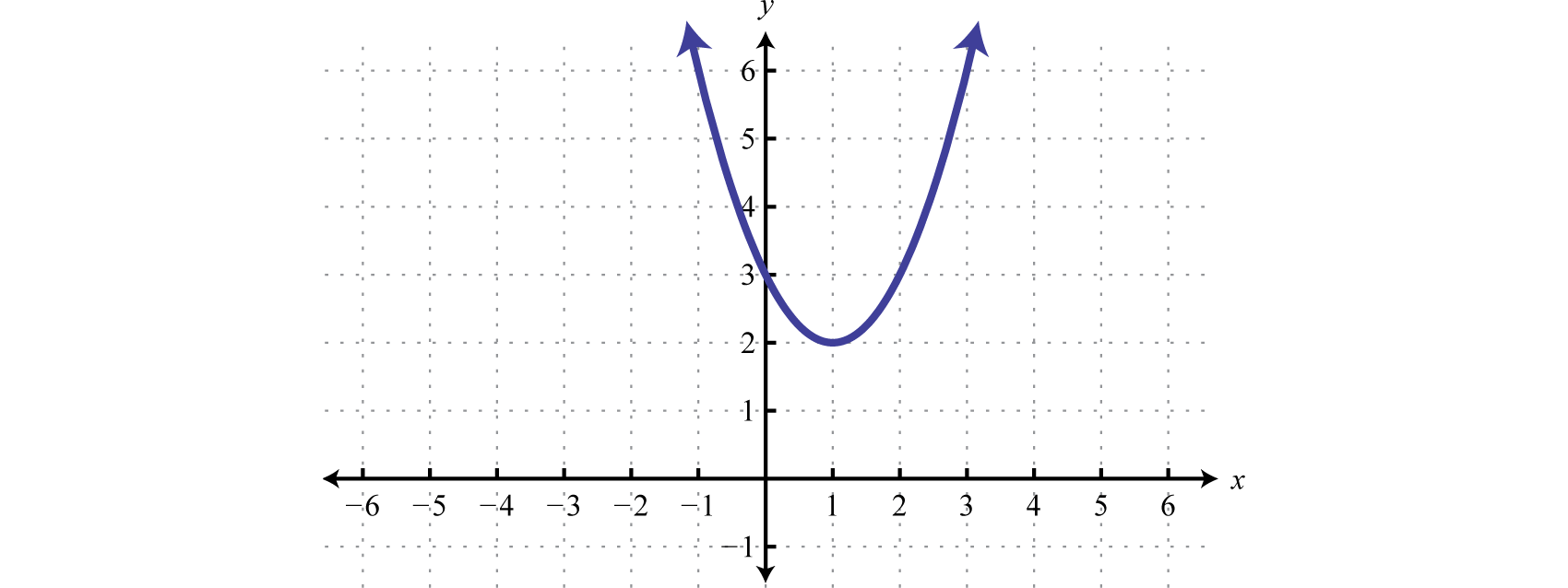We can see that it has no x-intercepts and is always above the x-axis (positive). If the question was to solve $x2−2x+3<0$, then the answer would have been no solution. The function is never negative.

Try this! Solve: $9x2−12x+4≤0.$

Answer: One solution, $23.$

### Example 7

Find the domain: $f(x)=x2−4.$

Solution:

Recall that the argument of a square root function must be nonnegative. Therefore, the domain consists of all real numbers for x such that $x2−4$ is greater than or equal to zero.

$x2−4≥0$

It should be clear that $x2−4=0$ has two solutions $x=±2$; these are the critical values. Choose test values in each interval and evaluate $f(x)=x2−4.$

$f(−3)=(−3)2−4=9−4=+f(0)=(0)2−4=0−4=−f(3)=(3)2−4=9−4=+$

Shade in the x-values that produce positive results.Answer: Domain: $( −∞,−2 ]∪[ 2,∞ )$

### Key Takeaways

• Quadratic inequalities can have infinitely many solutions, one solution or no solution.
• We can solve quadratic inequalities graphically by first rewriting the inequality in standard form, with zero on one side. Graph the quadratic function and determine where it is above or below the x-axis. If the inequality involves “less than,” then determine the x-values where the function is below the x-axis. If the inequality involves “greater than,” then determine the x-values where the function is above the x-axis.
• We can streamline the process of solving quadratic inequalities by making use of a sign chart. A sign chart gives us a visual reference that indicates where the function is above the x-axis using positive signs or below the x-axis using negative signs. Shade in the appropriate x-values depending on the original inequality.
• To make a sign chart, use the function and test values in each region bounded by the roots. We are only concerned if the function is positive or negative and thus a complete calculation is not necessary.

### Part A: Solutions to Quadratic Inequalities

Determine whether or not the given value is a solution.

1. $x2−x+1<0$; $x=−1$

2. $x2+x−1>0$; $x=−2$

3. $4x2−12x+9≤0$; $x=32$

4. $5x2−8x−4<0$; $x=−25$

5. $3x2−x−2≥0$; $x=0$

6. $4x2−x+3≤0$; $x=−1$

7. $2−4x−x2<0$; $x=12$

8. $5−2x−x2>0$; $x=0$

9. $−x2−x−9<0$; $x=−3$

10. $−x2+x−6≥0$; $x=6$

Given the graph of f determine the solution set.

1. $f(x)≤0$;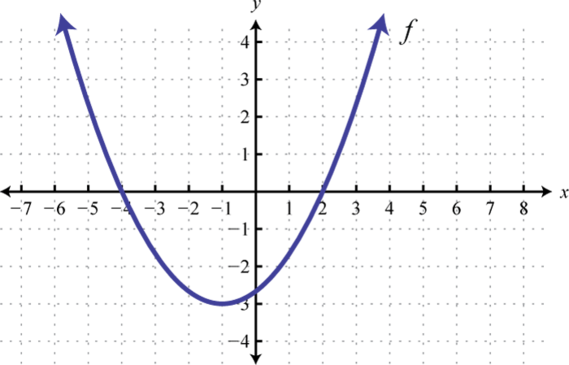2. $f(x)≥0$;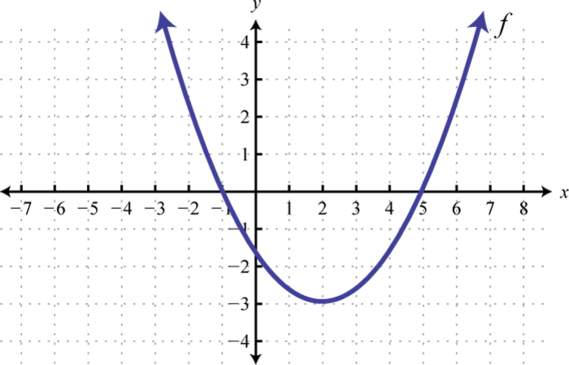3. $f(x)≥0$;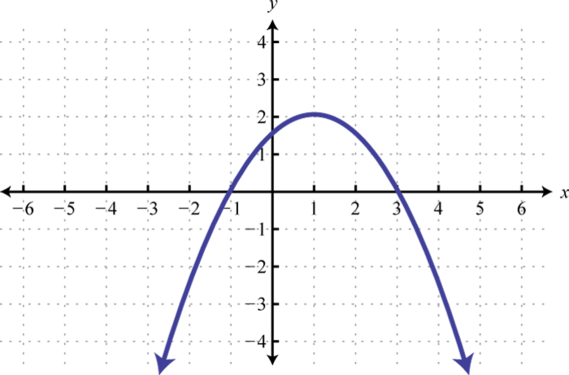4. $f(x)≤0$;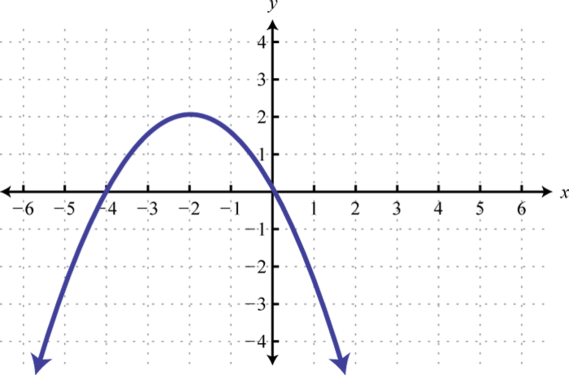5. $f(x)>0$;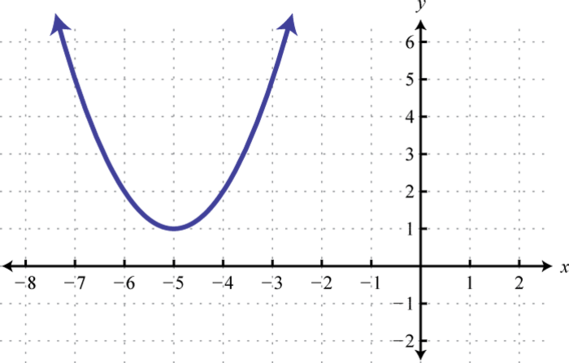6. $f(x)<0$;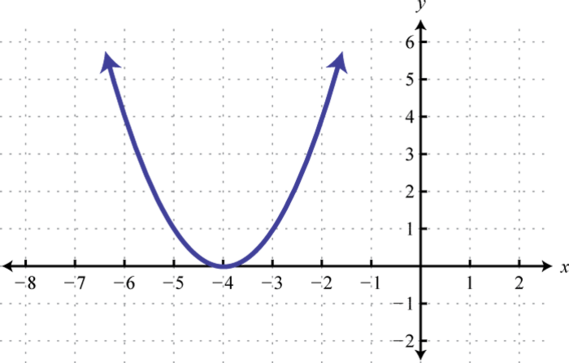7. $f(x)>0$;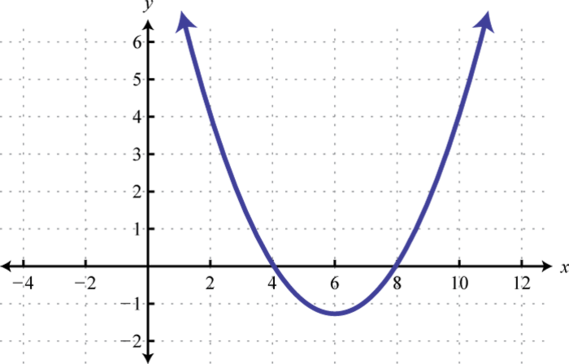8. $f(x)<0$;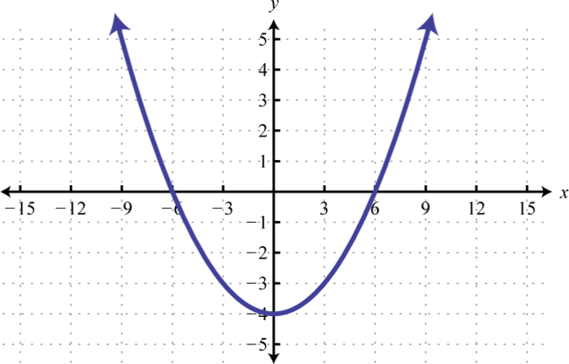9. $f(x)≥0$;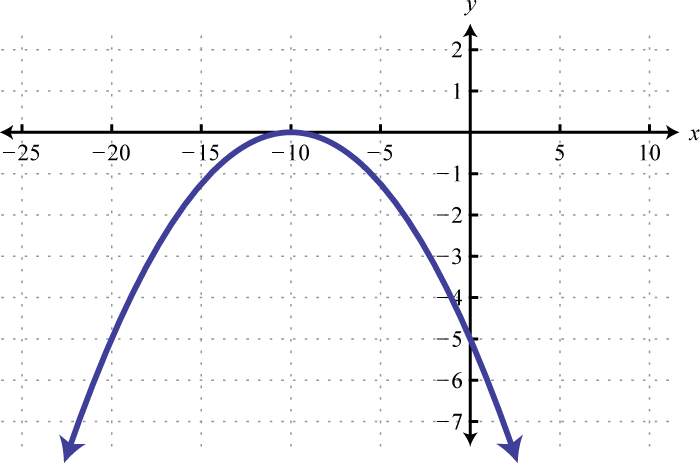10. $f(x)<0$;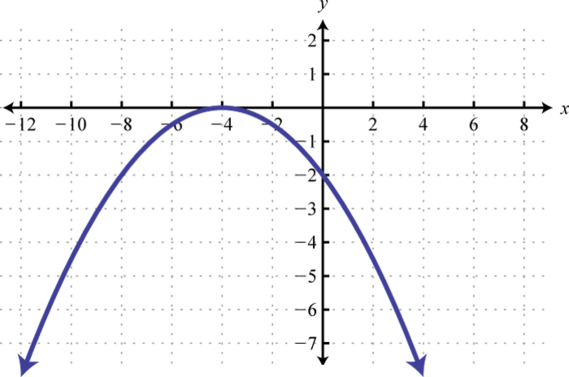Use the transformations to graph the following and then determine the solution set.

1. $x2−1>0$

2. $x2+2>0$

3. $(x−1)2>0$

4. $(x+2)2≤0$

5. $(x+2)2−1≤0$

6. $(x+3)2−4>0$

7. $−x2+4≥0$

8. $−(x+2)2>0$

9. $−(x+3)2+1<0$

10. $−(x−4)2+9>0$

### Part B: Solving Quadratic Inequalities

Use a sign chart to solve and graph the solution set. Present answers using interval notation.

1. $x2−x−12>0$

2. $x2−10x+16>0$

3. $x2+2x−24<0$

4. $x2+15x+54<0$

5. $x2−23x−24≤0$

6. $x2−12x+20≤0$

7. $2x2−11x−6≥0$

8. $3x2+17x−6≥0$

9. $8x2−18x−5<0$

10. $10x2+17x+6>0$

11. $9x2+30x+25≤0$

12. $16x2−40x+25≤0$

13. $4x2−4x+1>0$

14. $9x2+12x+4>0$

15. $−x2−x+30≥0$

16. $−x2−6x+27≤0$

17. $x2−64<0$

18. $x2−81≥0$

19. $4x2−9≥0$

20. $16x2−25<0$

21. $25−4x2≥0$

22. $1−49x2<0$

23. $x2−8>0$

24. $x2−75≤0$

25. $2x2+1>0$

26. $4x2+3<0$

27. $x−x2>0$

28. $3x−x2≤0$

29. $x2−x+1<0$

30. $x2+x−1>0$

31. $4x2−12x+9≤0$

32. $5x2−8x−4<0$

33. $3x2−x−2≥0$

34. $4x2−x+3≤0$

35. $2−4x−x2<0$

36. $5−2x−x2>0$

37. $−x2−x−9<0$

38. $−x2+x−6≥0$

39. $−2x2+4x−1≥0$

40. $−3x2−x+1≤0$

Find the domain of the function.

1. $f(x)=x2−25$

2. $f(x)=x2+3x$

3. $g(x)=3x2−x−2$

4. $g(x)=12x2−9x−3$

5. $h(x)=16−x2$

6. $h(x)=3−2x−x2$

7. $f(x)=x2+10$

8. $f(x)=9+x2$

9. A robotics manufacturing company has determined that its weekly profit in thousands of dollars is modeled by $P(n)=−n2+30n−200$ where n represents the number of units it produces and sells. How many units must the company produce and sell to maintain profitability. (Hint: Profitability occurs when profit is greater than zero.)

10. The height in feet of a projectile shot straight into the air is given by $h(t)=−16t2+400t$ where t represents the time in seconds after it is fired. In what time intervals is the projectile under 1,000 feet? Round to the nearest tenth of a second.

### Part C: Discussion Board

1. Does the sign chart for any given quadratic function always alternate? Explain and illustrate your answer with some examples.

2. Research and discuss other methods for solving a quadratic inequality.

3. Explain the difference between a quadratic equation and a quadratic inequality. How can we identify and solve each? What is the geometric interpretation of each?

1. No

2. Yes

3. No

4. Yes

5. Yes

6. $[−4,2]$

7. $[−1,3]$

8. $(−∞,∞)$

9. $(−∞,4)∪(8,∞)$

10. ${−10}$

11.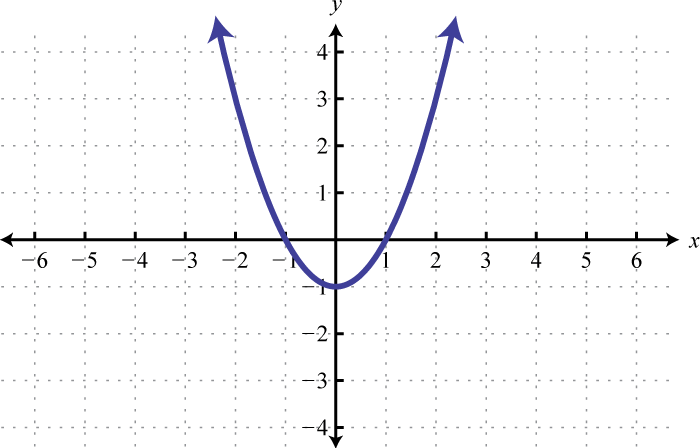$(−∞,−1)∪(1,∞)$

12.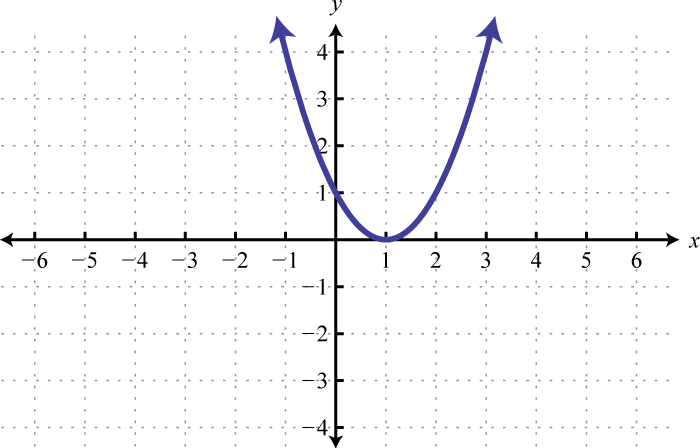$(−∞,1)∪(1,∞)$

13.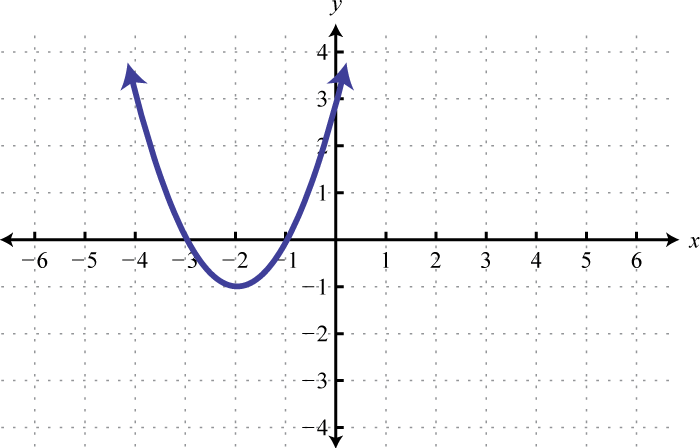$[−3,−1]$

14.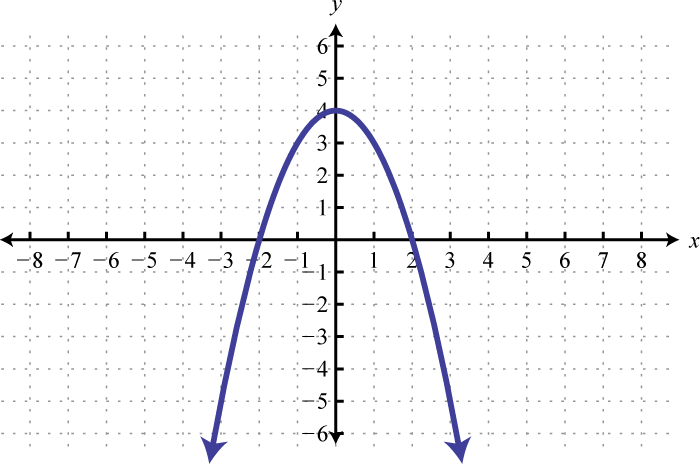$[−2,2]$

15.$(−∞,−4)∪(−2,∞)$

1. $(−∞,−3)∪(4,∞)$

2. $(−6,4)$

3. $[−1,24]$

4. $(−∞,−12]∪[6,∞)$
5. $(−14,52)$
6. $−53$
7. $(−∞,12)∪(12,∞)$
8. $[−6,5]$

9. $(−8,8)$

10. $(−∞,−32]∪[32,∞)$
11. $[−52,52]$
12. $(−∞,−22)∪(22,∞)$

13. $(−∞,∞)$

14. $(0,1)$

15. Ø

16. $32$

17. $(−∞,−23]∪[1,∞)$
18. $(−∞,−2−6)∪(−2+6,∞)$

19. $(−∞,∞)$

20. $[2−22,2+22]$
21. $(−∞,−5]∪[5,∞)$
22. $(−∞,−23]∪[1,∞)$
23. $[−4,4]$

24. $(−∞,∞)$

25. The company must produce and sell more than 10 units and fewer than 20 units each week.

## 6.6 Solving Polynomial and Rational Inequalities

### Learning Objectives

1. Solve polynomial inequalities.
2. Solve rational inequalities.

## Solving Polynomial Inequalities

A polynomial inequalityA mathematical statement that relates a polynomial expression as either less than or greater than another. is a mathematical statement that relates a polynomial expression as either less than or greater than another. We can use sign charts to solve polynomial inequalities with one variable.

### Example 1

Solve: $x(x+3)2(x−4)<0.$

Solution:

Begin by finding the critical numbers. For a polynomial inequality in standard form, with zero on one side, the critical numbers are the roots. Because $f(x)=x(x+3)2(x−4)$ is given in its factored form the roots are apparent. Here the roots are: 0, −3, and 4. Because of the strict inequality, plot them using open dots on a number line.In this case, the critical numbers partition the number line into four regions. Test values in each region to determine if f is positive or negative. Here we choose test values −5, −1, 2, and 6. Remember that we are only concerned with the sign (+ or −) of the result.

$f(−5)=(−5)(−5+3)2(−5−4)=(−)(−)2(−)=+Positivef(−1)=(−1)(−1+3)2(−1−4)=(−)(+)2(−)=+Positivef(2)=(2)(2+3)2(2−4)=(+)(+)2(−)=−Negativef(6)=(6)(6+3)2(6−4)=(+)(+)2(+)=+Positive$

After testing values we can complete a sign chart.The question asks us to find the values where $f(x)<0$, or where the function is negative. From the sign chart we can see that the function is negative for x-values in between 0 and 4.We can express this solution set in two ways:

${x|0

In this textbook we will continue to present solution sets using interval notation.

Answer: $(0,4)$

Graphing polynomials such as the one in the previous example is beyond the scope of this textbook. However, the graph of this function is provided below. Compare the graph to its corresponding sign chart.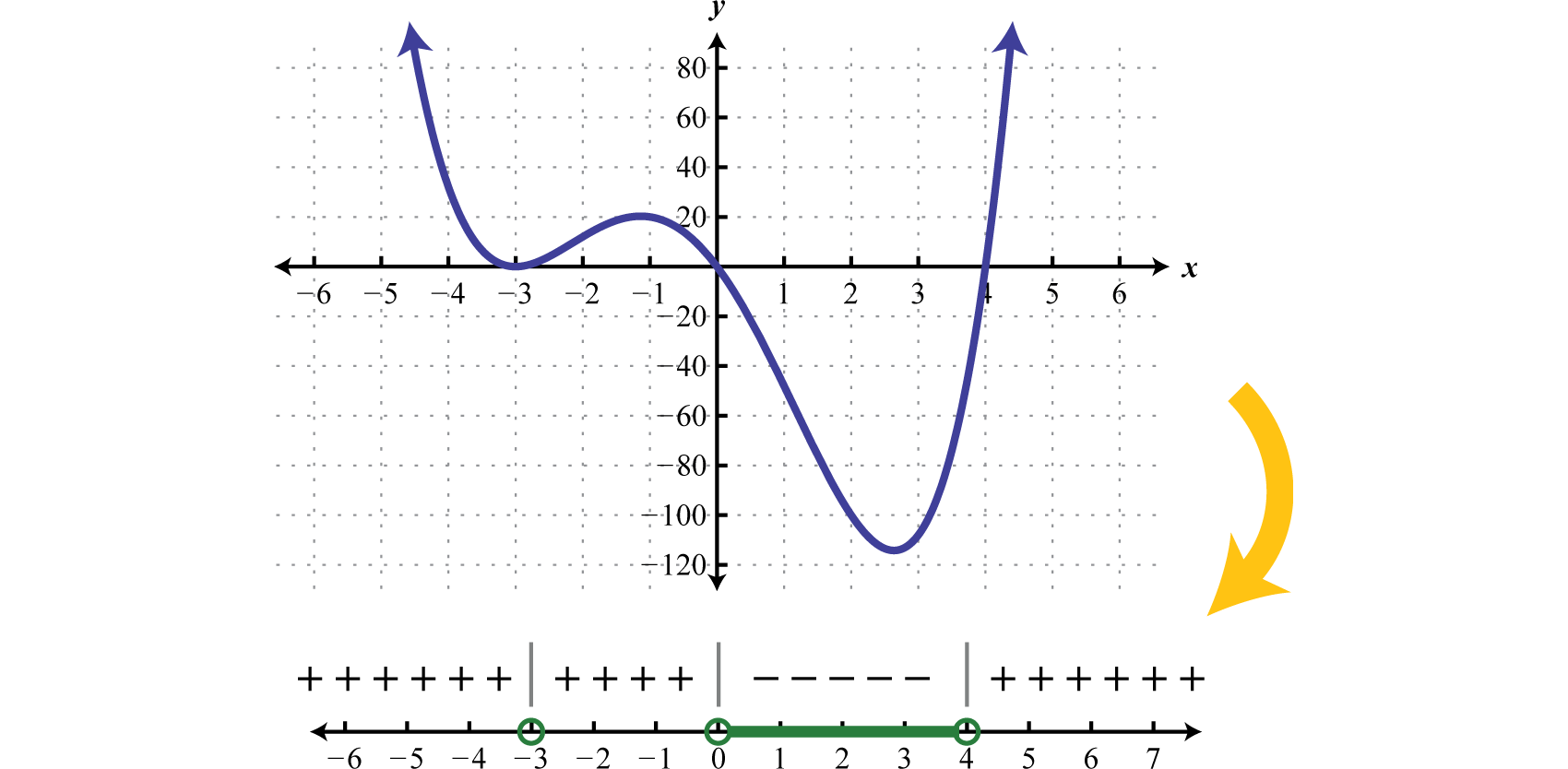Certainly it may not be the case that the polynomial is factored nor that it has zero on one side of the inequality. To model a function using a sign chart, all of the terms should be on one side and zero on the other. The general steps for solving a polynomial inequality are listed in the following example.

### Example 2

Solve: $2x4>3x3+9x2.$

Solution:

Step 1: Obtain zero on one side of the inequality. In this case, subtract to obtain a polynomial on the left side in standard from.

$2x4>3x3+9x22x4−3x3−9x2>0$

Step 2: Find the critical numbers. Here we can find the zeros by factoring.

$2x4−3x3−9x2=0x2(2x2−3x−9)=0x2(2x+3)(x−3)=0$

There are three solutions, hence, three critical numbers $−32$, 0, and 3.The strict inequality indicates that we should use open dots.Step 3: Create a sign chart. In this case use $f(x)=x2(2x+3)(x−3)$ and test values −2, −1, 1, and 4 to determine the sign of the function in each interval.

$f(−2)=(−2)2[2(−2)+3](−2−3)=(−)2(−)(−)=+f(−1)=(−1)2[2(−1)+3](−1−3)=(−)2(+)(−)=−f(1)=(1)2[2(1)+3](1−3)=(+)2(+)(−)=−f(4)=(4)2[2(4)+3](4−3)=(+)2(+)(+)=+$

With this information we can complete the sign chart.Step 4: Use the sign chart to answer the question. Here the solution consists of all values for which $f(x)>0.$ Shade in the values that produce positive results and then express this set in interval notation.Answer: $(−∞,−32)∪(3,∞)$

### Example 3

Solve: $x3+x2≤4(x+1).$

Solution:

Begin by rewriting the inequality in standard form, with zero on one side.

$x3+x2≤4(x+1)x3+x2≤4x+4x3+x2−4x−4≤0$

Next find the critical numbers of $f(x)=x3+x2−4x−4$:

$x3+x2−4x−4=0Factor by grouping.x2(x+1)−4(x+1)=0(x+1)(x2−4)=0(x+1)(x+2)(x−2)=0$

The critical numbers are −2, −1, and 2. Because of the inclusive inequality ($≤$) we will plot them using closed dots.Use test values −3, $−32$, 0, and 3 to create a sign chart.

$f(−3)=(−3+1)(−3+2)(−3−2)=(−)(−)(−)=−f(−32)=(−32+1)(−32+2)(−32−2)=(−)(+)(−)=+f(0)=(0+1)(0+2)(0−2)=(+)(+)(−)=−f(3)=(3+1)(3+2)(3−2)=(+)(+)(+)=+$

And we haveUse the sign chart to shade in the values that have negative results ($f(x)≤0$).Answer: $(−∞,−2]∪[−1,2]$

Try this! Solve: $−3x4+12x3−9x2>0.$

Answer: $(1,3)$

## Solving Rational Inequalities

A rational inequalityA mathematical statement that relates a rational expression as either less than or greater than another. is a mathematical statement that relates a rational expression as either less than or greater than another. Because rational functions have restrictions to the domain we must take care when solving rational inequalities. In addition to the zeros, we will include the restrictions to the domain of the function in the set of critical numbers.

### Example 4

Solve: $(x−4)(x+2)(x−1)≥0.$

Solution:

The zeros of a rational function occur when the numerator is zero and the values that produce zero in the denominator are the restrictions. In this case,

$Roots (Numerator)Restriction (Denominator)x−4=0orx+2=0x=4x=−2x−1=0x=1$

Therefore the critical numbers are −2, 1, and 4. Because of the inclusive inequality ($≥$) use a closed dot for the roots {−2, 4} and always use an open dot for restrictions {1}. Restrictions are never included in the solution set.Use test values $x=−4,0,2,6.$

$f(−4)=(−4−4)(−4+2)(−4−1)=(−)(−)(−)=−f(0)=(0−4)(0+2)(0−1)=(−)(+)(−)=+f(2)=(2−4)(2+2)(2−1)=(−)(+)(+)=−f(6)=(6−4)(6+2)(6−1)=(+)(+)(+)=+$

And then complete the sign chart.The question asks us to find the values for which $f(x)≥0$, in other words, positive or zero. Shade in the appropriate regions and present the solution set in interval notation.Answer: $[−2,1)∪[4,∞)$

Graphing such rational functions like the one in the previous example is beyond the scope of this textbook. However, the graph of this function is provided below. Compare the graph to its corresponding sign chart.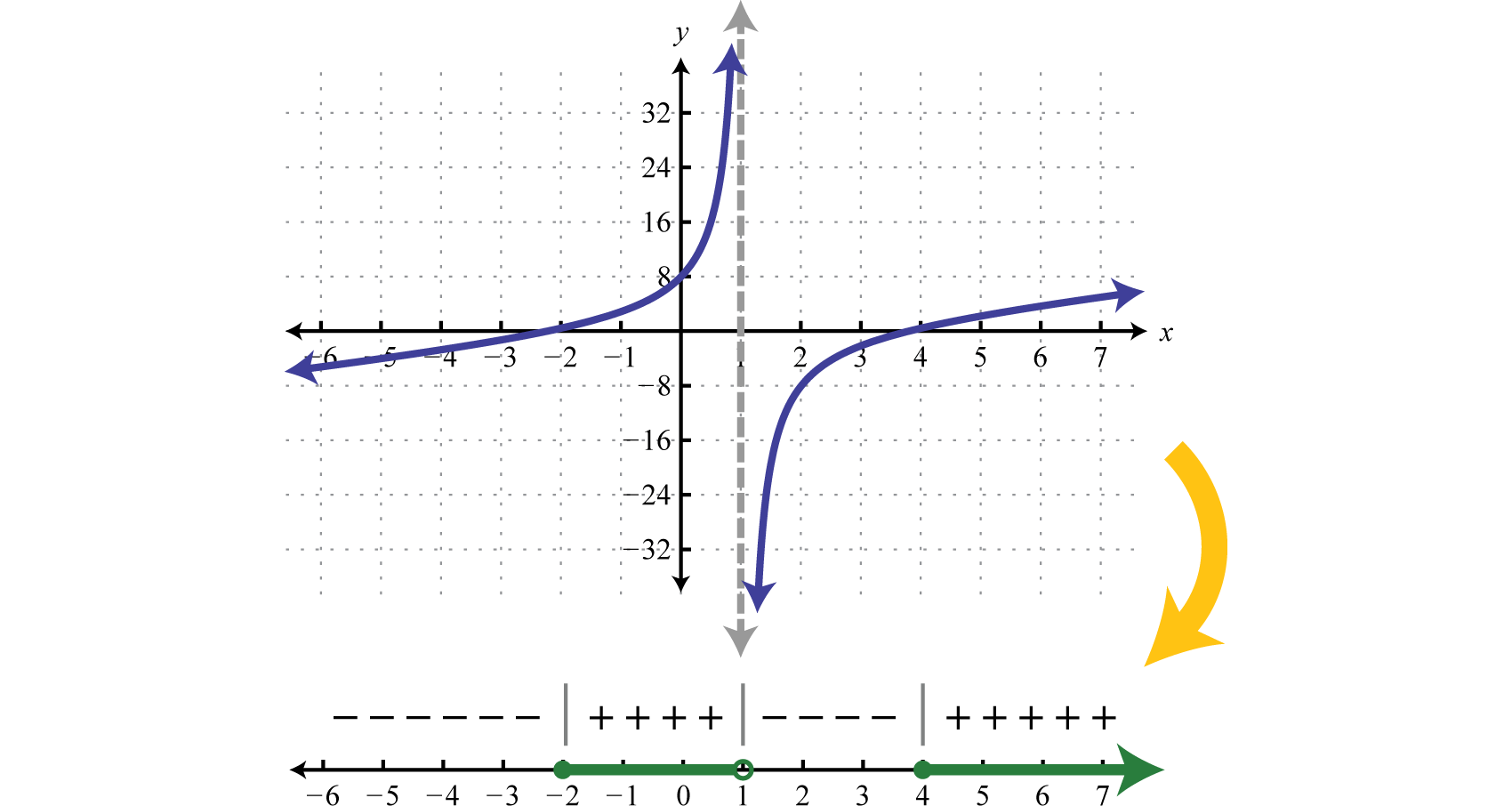Notice that the restriction $x=1$ corresponds to a vertical asymptote which bounds regions where the function changes from positive to negative. While not included in the solution set, the restriction is a critical number. Before creating a sign chart we must ensure the inequality has a zero on one side. The general steps for solving a rational inequality are outlined in the following example.

### Example 5

Solve: $7x+3<2.$

Solution:

Step 1: Begin by obtaining zero on the right side.

$7x+3<27x+3−2<0$

Step 2: Determine the critical numbers. The critical numbers are the zeros and restrictions. Begin by simplifying to a single algebraic fraction.

$7x+3−21<07−2(x+3)x+3<07−2x−6x+3<0−2x+1x+3<0$

Next find the critical numbers. Set the numerator and denominator equal to zero and solve.

$RootRestriction−2x+1=0−2x=−1x=12x+3=0x=−3$

In this case, the strict inequality indicates that we should use an open dot for the root.Step 3: Create a sign chart. Choose test values −4, 0, and 1.

$f(−4)=−2(−4)+1−4+3=+−=−f(0)=−2(0)+10+3=++=+f(1)=−2(1)+11+3=−+=−$

And we haveStep 4: Use the sign chart to answer the question. In this example we are looking for the values for which the function is negative, $f(x)<0.$ Shade the appropriate values and then present your answer using interval notation.Answer: $(−∞,−3)∪(12,∞)$

### Example 6

Solve: $1x2−4≤12−x$

Solution:

Begin by obtaining zero on the right side.

$1x2−4≤12−x1x2−4−12−x≤0$

Next simplify the left side to a single algebraic fraction.

$1x2−4−12−x≤01(x+2)(x−2)−1−(x−2)≤01(x+2)(x−2)+1(x+2)(x−2)(x+2)≤01+x+2(x+2)(x−2)≤0x+3(x+2)(x−2)≤0$

The critical numbers are −3, −2, and 2. Note that ±2 are restrictions and thus we will use open dots when plotting them on a number line. Because of the inclusive inequality we will use a closed dot at the root −3.Choose test values −4, $−212=−52$, 0, and 3.

$f(−4)=−4+3(−4+2)(−4−2)=(−)(−)(−)=−f(−52)=−52+3(−52+2)(−52−2)=(+)(−)(−)=+f(0)=0+3(0+2)(0−2)=(+)(+)(−)=−f(3)=3+3(3+2)(3−2)=(+)(+)(+)=+$

Construct a sign chart.Answer the question; in this case, find x where $f(x)≤0.$Answer: $(−∞,−3]∪(−2,2)$

Try this! Solve: $2x22x2+7x−4≥xx+4.$

Answer: $(−4,0]∪(12,∞)$

### Key Takeaways

• When a polynomial inequality is in standard form, with zero on one side, the roots of the polynomial are the critical numbers. Create a sign chart that models the function and then use it to answer the question.
• When a rational inequality is written as a single algebraic fraction, with zero on one side, the roots as well as the restrictions are the critical numbers. The values that produce zero in the numerator are the roots, and the values that produce zero in the denominator are the restrictions. Always use open dots for restrictions, regardless of the given inequality, because restrictions are not part of the domain. Create a sign chart that models the function and then use it to answer the question.

### Part A: Solving Polynomial Inequalities

Solve. Present answers using interval notation.

1. $x(x+1)(x−3)>0$

2. $x(x−1)(x+4)<0$

3. $(x+2)(x−5)2<0$

4. $(x−4)(x+1)2≥0$

5. $(2x−1)(x+3)(x+2)≤0$

6. $(3x+2)(x−4)(x−5)≥0$

7. $x(x+2)(x−5)2<0$

8. $x(2x−5)(x−1)2>0$

9. $x(4x+3)(x−1)2≥0$

10. $(x−1)(x+1)(x−4)2<0$

11. $(x+5)(x−10)(x−5)2≥0$

12. $(3x−1)(x−2)(x+2)2≤0$

13. $−4x(4x+9)(x−8)2>0$

14. $−x(x−10)(x+7)2>0$

Solve.

1. $x3+2x2−24x≥0$

2. $x3−3x2−18x≤0$

3. $4x3−22x2−12x<0$

4. $9x3+30x2−24x>0$

5. $12x4+44x3>80x2$

6. $6x4+12x3<48x2$

7. $x(x2+25)<10x2$

8. $x3>12x(x−3)$

9. $x4−5x2+4≤0$

10. $x4−13x2+36≥0$

11. $x4>3x2+4$

12. $4x4<3−11x2$

13. $9x3−3x2−81x+27≤0$

14. $2x3+x2−50x−25≥0$

15. $x3−3x2+9x−27>0$

16. $3x3+5x2+12x+20<0$

### Part B: Solving Rational Inequalities

Solve.

1. $xx−3>0$
2. $x−5x>0$
3. $(x−3)(x+1)x<0$
4. $(x+5)(x+4)(x−2)<0$
5. $(2x+1)(x+5)(x−3)(x−5)≤0$
6. $(3x−1)(x+6)(x−1)(x+9)≥0$
7. $(x−8)(x+8)−2x(x−2)≥0$
8. $(2x+7)(x+4)x(x+5)≤0$
9. $x2(2x+3)(2x−3)≤0$
10. $(x−4)2−x(x+1)>0$
11. $−5x(x−2)2(x+5)(x−6)≥0$
12. $(3x−4)(x+5)x(x−4)2≥0$
13. $1(x−5)4>0$
14. $1(x−5)4<0$

Solve.

1. $x2−11x−12x+4<0$
2. $x2−10x+24x−2>0$
3. $x2+x−302x+1≥0$
4. $2x2+x−3x−3≤0$
5. $3x2−4x+1x2−9≤0$
6. $x2−162x2−3x−2≥0$
7. $x2−12x+20x2−10x+25>0$
8. $x2+15x+36x2−8x+16<0$
9. $8x2−2x−12x2−3x−14≤0$
10. $4x2−4x−15x2+4x−5≥0$
11. $1x+5+5x−1>0$
12. $5x+4−1x−4<0$
13. $1x+7>1$
14. $1x−1<−5$
15. $x≥30x−1$
16. $x≤1−2xx−2$
17. $1x−1≤2x$
18. $3x+1>−1x$
19. $4x−3≤1x+3$
20. $2x−9x+49x−8<0$
21. $x2(x+2)−1x+2≤12x(x+2)$
22. $12x+1−92x−1>2$
23. $3xx2−4−2x−2<0$
24. $x2x+1+42x2−7x−4<0$
25. $x+12x2+5x−3 ≥x4x2−1$
26. $x2−142x2−7x−4≤51+2x$

### Part C: Discussion Board

1. Does the sign chart for any given polynomial or rational function always alternate? Explain and illustrate your answer with some examples.